# KR100681984B1 - Multi-carrier cdma communication device, multi-carrier cdma transmitting device, and multi-carrier cdma receiving device - Google Patents

Multi-carrier cdma communication device, multi-carrier cdma transmitting device, and multi-carrier cdma receiving device Download PDF

## Info

Publication number
KR100681984B1
KR100681984B1 KR1020027003844A KR20027003844A KR100681984B1 KR 100681984 B1 KR100681984 B1 KR 100681984B1 KR 1020027003844 A KR1020027003844 A KR 1020027003844A KR 20027003844 A KR20027003844 A KR 20027003844A KR 100681984 B1 KR100681984 B1 KR 100681984B1
Authority
KR
South Korea
Prior art keywords
subcarrier
signal
means
frequency
units
Prior art date
Application number
KR1020027003844A
Other languages
Korean (ko)
Other versions
KR20020035149A (en
Inventor
노부히사 가따오까
히로야스 사노
Original Assignee
미쓰비시덴키 가부시키가이샤
Priority date (The priority date is an assumption and is not a legal conclusion. Google has not performed a legal analysis and makes no representation as to the accuracy of the date listed.)
Filing date
Publication date
Priority to JPJP-P-2000-00226027 priority Critical
Priority to JP2000226027 priority
Priority to JP2000259858 priority
Priority to JPJP-P-2000-00259858 priority
Application filed by 미쓰비시덴키 가부시키가이샤 filed Critical 미쓰비시덴키 가부시키가이샤
Publication of KR20020035149A publication Critical patent/KR20020035149A/en
Application granted granted Critical
Publication of KR100681984B1 publication Critical patent/KR100681984B1/en

• 238000004891 communication Methods 0.000 title claims description 70
• 230000000051 modifying Effects 0.000 claims abstract description 137
• 238000000034 methods Methods 0.000 claims abstract description 55
• 230000000875 corresponding Effects 0.000 claims description 59
• 239000000562 conjugates Substances 0.000 claims description 42
• 230000001360 synchronised Effects 0.000 claims description 40
• 230000002194 synthesizing Effects 0.000 claims description 31
• 238000006243 chemical reaction Methods 0.000 claims description 18
• 230000000694 effects Effects 0.000 description 13
• 230000001629 suppression Effects 0.000 description 7

## Images

•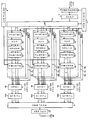•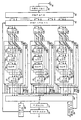•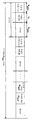•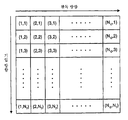•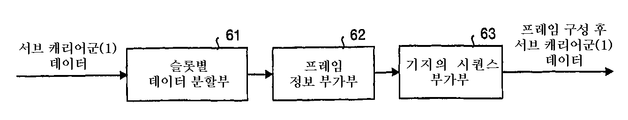•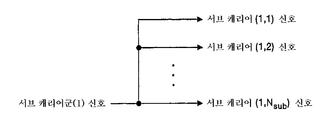•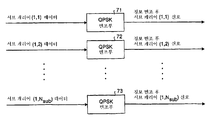•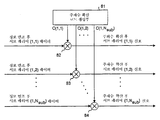•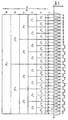•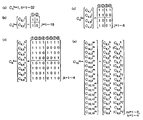••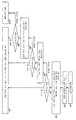••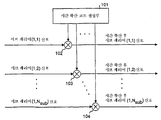•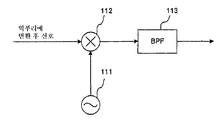•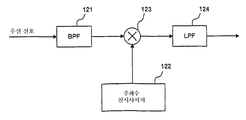•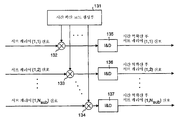•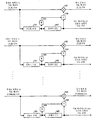•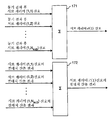••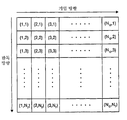••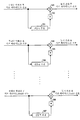••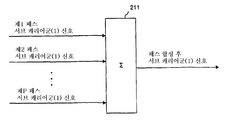••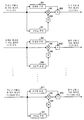•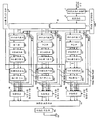•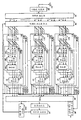•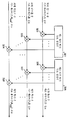•••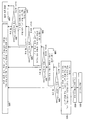••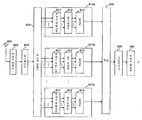••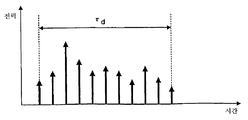••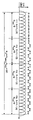## Classifications

• HELECTRICITY
• H04ELECTRIC COMMUNICATION TECHNIQUE
• H04JMULTIPLEX COMMUNICATION
• H04J13/00Code division multiplex systems
• H04J13/16Code allocation
• H04J13/18Allocation of orthogonal codes
• H04J13/20Allocation of orthogonal codes having an orthogonal variable spreading factor [OVSF]
• HELECTRICITY
• H04ELECTRIC COMMUNICATION TECHNIQUE
• H04BTRANSMISSION
• H04B1/00Details of transmission systems, not covered by a single one of groups H04B3/00 - H04B13/00; Details of transmission systems not characterised by the medium used for transmission
• H04B1/692Hybrid techniques using combinations of two or more spread spectrum techniques
• HELECTRICITY
• H04ELECTRIC COMMUNICATION TECHNIQUE
• H04JMULTIPLEX COMMUNICATION
• H04J13/00Code division multiplex systems
• H04J13/0007Code type
• H04J13/004Orthogonal
• H04J13/0044OVSF [orthogonal variable spreading factor]
• HELECTRICITY
• H04ELECTRIC COMMUNICATION TECHNIQUE
• H04LTRANSMISSION OF DIGITAL INFORMATION, e.g. TELEGRAPHIC COMMUNICATION
• H04L5/00Arrangements affording multiple use of the transmission path
• H04L5/02Channels characterised by the type of signal
• H04L5/023Multiplexing of multicarrier modulation signals
• H04L5/026Multiplexing of multicarrier modulation signals using code division
• HELECTRICITY
• H04ELECTRIC COMMUNICATION TECHNIQUE
• H04BTRANSMISSION
• H04B1/00Details of transmission systems, not covered by a single one of groups H04B3/00 - H04B13/00; Details of transmission systems not characterised by the medium used for transmission
• H04B1/707Spread spectrum techniques using direct sequence modulation
• HELECTRICITY
• H04ELECTRIC COMMUNICATION TECHNIQUE
• H04BTRANSMISSION
• H04B1/00Details of transmission systems, not covered by a single one of groups H04B3/00 - H04B13/00; Details of transmission systems not characterised by the medium used for transmission
• H04B1/707Spread spectrum techniques using direct sequence modulation
• H04B1/7097Interference-related aspects
• H04B1/711Interference-related aspects the interference being multi-path interference
• H04B1/7115Constructive combining of multi-path signals, i.e. RAKE receivers
• H04B1/712Weighting of fingers for combining, e.g. amplitude control or phase rotation using an inner loop

## Abstract

A data / modulation control unit 4 which sets a predetermined parameter for demodulation processing, and a subcarrier group modulation processing unit 6a to which frequency spreading processing is performed in subcarrier signal units of the subcarrier group based on the setting. 8c) and time spreading units 10a to 40c which multiplex all the signals after the modulation process and the frequency spreading process in units of subcarrier signals, and perform time spreading processing on the multiplexed signal, and the receiving side includes subcarriers. Time despreading units 35a to 35c for performing time despreading in signal units, and subcarrier group demodulation processing units 36a to 38c for performing frequency despreading in units of subcarrier signals after the time despreading process. It is set as a configuration.

## Description

MULTI-CARRIER CDMA COMMUNICATION DEVICE, MULTI-CARRIER CDMA TRANSMITTING DEVICE, AND MULTI-CARRIER CDMA RECEIVING DEVICE}

BACKGROUND OF THE INVENTION 1. Field of the Invention The present invention relates to a communication apparatus of a mobile communication system employing a multiple access method using a multicarrier CDMA system. In particular, a multicarrier CDMA communication capable of obtaining good bit error rate characteristics even in a communication environment affected by frequency selective paging. A device, a multicarrier CDMA transmitter and a multicarrier CDMA receiver.

The conventional multicarrier CDMA communication apparatus will be described below. As a communication apparatus of a mobile communication system employing a multiple access method using a multicarrier CDMA method, for example, the document "SC / DS-CDMA, MC / DS-CDMA, MC-CDMA in downlink broadband wireless packet transmission". Comparison of Methods, Korea Information and Communications Society RCS99-130 p. 63-70 October 1999, "Overview of Multicarrier CDMA, IEEE Communications Magazine p. 126-133 December 1997 ".

Here, the configuration and operation of the conventional multicarrier CDMA communication apparatus will be described with reference to the drawings. FIG. 34 is a diagram showing the configuration of a conventional multicarrier CDMA transmitter (hereinafter simply referred to as a transmitter), and FIG. 35 is a diagram showing the configuration of a conventional multicarrier CDMA receiver (hereinafter, simply referred to as a receiver). to be.

In Fig. 34, reference numeral 501 denotes a convolutional encoder, reference numeral 502 denotes an interleaver, reference numeral 503 denotes a serial / parallel conversion unit (hereinafter referred to as S / P), and reference numeral 510a. , 510b, 510c are first, second, and N scg subcarrier group modulation processing units, reference numeral 511 is a frame creation unit, reference numeral 512 is a copy unit, and reference numeral 513 is An information modulator, reference numeral 514 is a frequency spreader, reference numerals 504a, 504b, and 504c are multiplexers, reference numeral 505 is an inverse Fourier transform, and reference numeral 506 is a guard interval. (GI) addition unit, reference numeral 507 is a frequency converter, and reference numeral 508 is an antenna.

35, reference numeral 601 is an antenna, reference numeral 602 is a frequency converter, reference numeral 603 is a guard interval (GI) removal unit, and reference numeral 604 is a Fourier transform unit. Reference numerals 610a, 610b, and 610c denote first, second, and N scg subcarrier demodulation processing units, reference numeral 611 denotes a frequency despreading unit, and reference numeral 612 denotes a synchronous detector. Reference numeral 613 is a combining unit, reference numeral 605 is a parallel / serial conversion unit (hereinafter referred to as P / S), reference numeral 606 is a deinterleaver unit, and reference numeral 607. Is the Viterbi decoder.

36 is a diagram illustrating a format of a transmission slot on a subcarrier basis. As such, the transmission slot is composed of a pilot symbol portion (base sequence) and a data portion.

37 is a figure which shows an example of the impulse response of a frequency selective paging transmission path. For example, in a mobile communication system, radio waves are reflected, diffracted, and scattered by surrounding buildings and terrain, and thus, incoming waves (multi-pass waves) that pass through a plurality of transmission paths interfere with each other. An impulse response to the selective paging transmission occurs.

34 and 35, the operation of the conventional multicarrier CDMA communication apparatus will be described. In this example, data transmission and reception by a base station and a plurality of terminals are assumed. First, the operation of the transmission device will be described.

For example, the convolutional coding unit 501 that has received transmission data for an arbitrary terminal generates coded data according to a predetermined coding rate. This coded data is written in the vertical direction to the interleaver 502 having a block having a vertical size of Nr (predetermined integer) and a horizontal size of Nc (predetermined integer), and also in the horizontal direction. Is read. In other words, the interleaver 502 outputs the rearranged signal as encoded data.

The S / P 503 that receives the encoded data converts the data into N scg (predetermined integer) parallel data, and outputs the converted output to the subcarrier group modulation processing units 510a, 510b, ..., 510c, respectively. Output In addition, since the same signal processing is performed in each of the first to Nth scgth subcarrier modulation processing units performing modulation processing in units of subcarrier groups, the operation of the first subcarrier group modulation processing unit 510a is performed here. Will be described only, and the description of other subcarrier group modulation processing units will be omitted.

The multiplexer 504a multiplexes the signals of the same number of subcarriers from other users with respect to the signals of the number of subcarriers after spreading the frequency to generate a multiplexed signal.

The inverse Fourier transform unit 505 performs inverse Fourier transform using the N scg × N sub subcarrier signals obtained from the multiplexers 504a, 504b, and 504c.

The guard interval adding unit 506 copies the rear part of the symbol in the signal after the inverse Fourier transform only for tau GI time and adds the copy part to the head of the symbol. 38 is a diagram illustrating a process of the guard interval adding unit 506. Here, τ GI is generally set to be larger than delay wave spread τ GI on the transmission path shown in FIG. 37.

Finally, the frequency converter 507 multiplies the carrier signal of the frequency oscillator (not shown) output with respect to the signal after the guard interval addition, and performs band limitation by using a band pass filter (not shown). And a transmission signal, and then output the transmission signal to the transmission path through the antenna 508. 39 is a diagram illustrating a transmission signal expressed on a frequency axis.

On the other hand, the receiving device receives the transmission signal affected by the frequency selective paging, etc. through the antenna 601, and the frequency converter 602 applies a band limit by a band pass filter (not shown) for the received signal. After that, a signal synchronized with a carrier frequency output by a frequency synthesizer (not shown) is multiplied by the signal after the band limitation is performed. In addition, only the low frequency components are filtered by the low pass filter (not shown), and the signal after multiplication is output as a signal after frequency conversion.

The guard interval removing unit 603 removes the guard interval and outputs a signal continuously connected in units of symbols. The Fourier transform unit 604, which has received the signal after the guard interval removal, performs the Fourier transform and outputs N scg x N sub subcarrier signals. Each subcarrier signal is first, second, ... to perform demodulation processing in units of subcarrier groups. , N scg th subcarrier group demodulation processing units 610a, 610b, and 610c. In addition, since the first, second, and N scg- th subcarrier demodulation processing units 610a, 610b, and 610c each perform the same signal processing, only the operation of the first subcarrier group demodulation processing unit 610a is performed here. The explanation is omitted for the other subcarrier group demodulation processing section.

The subcarrier group demodulation processing unit 610a receives the first N sub subcarrier signals. First, the frequency despreader 611 multiplies the individually allocated spreading codes by the N sub subcarrier signals. The despreading process is performed.

The synchronization detector 612, which has received each subcarrier signal after frequency despreading, estimates a transmission path using known sequence symbols added in units of frames and performs synchronous detection. That is, the synchronization detector 612 first calculates transmission path estimates in subcarrier units by synchronously adding N pilot known sequence symbols in a frame. Subsequently, the complex conjugate value and absolute value of the calculation result are calculated, and the complex conjugate value is divided by the absolute value, thereby extracting the phase component in units of subcarriers. Finally, the synchronous detection is performed by multiplying the subcarrier signal after the frequency despreading by multiplying the phase component in units of subcarriers.

The combining unit 613 adds all the subcarrier signals received after the synchronous detection, and calculates the first subcarrier group signal.

The P / S 605 receives subcarrier group signals from all subcarrier group demodulation processing units and converts them into serial signals. The serial signal is written in the horizontal direction to the deinterleaver 606 having a block having a vertical size of Nr (predetermined integer) and a horizontal size of Nc (predetermined integer), and further read in the vertical direction. do.

Finally, the Viterbi decoding unit 607 performs known Viterbi decoding on the received rearranged signal.

As described above, in the conventional multi-carrier CDMA communication apparatus, even when subjected to frequency-selective paging in which the amplitude and phase of the received wave are randomly changed, for example, the guard interval is set so that delay wave is included. In addition, by allocating a frequency spreading code on a user basis or on a channel basis, good bit error rate characteristics are obtained.

However, in the above-described conventional multicarrier CDMA communication apparatus, the spread of delay waves is large depending on the transmission path situation and may not be included in the guard interval. In such a case, there is a problem that the device is affected by frequency selective paging, and the influence of interference in the symbol becomes large, so that a good bit error rate characteristic cannot be obtained.

In addition, in the conventional multi-carrier CDMA communication apparatus, even when the level of the delayed wave is larger than that of the preceding wave, the delayed wave is utilized to increase the received signal quality (for example, signal power to interference power ratio (SIR)). There was a problem.

Moreover, in multimedia mobile communication, it is thought that the information rate needs to be adaptively changed according to the application to be handled. In other words, when the frequency spreading code is allocated to a user unit or a channel unit to be used, it is necessary to allocate a channel according to the information transmission rate. However, in the conventional multicarrier CDMA communication apparatus, since the frequency spreading codes need to be orthogonal to each other and the frequency spreading rate is fixed, there is a problem that the information rate cannot be adaptively changed.

In the conventional multi-carrier CDMA communication apparatus, when handover is performed between sectors and between cells so that communication is not interrupted upon reception of the mobile station (terminal), it is necessary to change the frequency to be used on the transmission path. There was a problem that the frequency utilization efficiency is reduced.

In addition, in the conventional multi-carrier CDMA communication apparatus, in order to solve the problem of keeping the reception quality of the mobile station constant regardless of the perspective from the base station, transmission power control is required on the base station side. The degree to which the frequency selective paging is affected is very different. Therefore, in the conventional system of keeping the received signal power constant in the mobile station, there is a problem that the received signal quality cannot be kept constant while suppressing the influence on other mobile stations (terminals).

Accordingly, the present invention provides a multi-carrier CDMA communication apparatus capable of realizing good bit error rate characteristics even when the spread of delay waves is not included in the guard interval and the transmission signal from the base station is affected by the frequency selective paging on the transmission path. It aims to do it.

Further, an object of the present invention is to provide a multi-carrier CDMA communication apparatus which can realize an improvement in received signal quality when the delayed wave is higher in level than the preceding wave.

Another object of the present invention is to provide a multi-carrier CDMA communication apparatus capable of changing the frequency spreading rate while maintaining orthogonal states of frequency spreading codes allocated on a user basis or a channel basis.

Further, an object of the present invention is to provide a multi-carrier CDMA communication apparatus capable of performing soft handover between sectors or between cells at the time of reception, without interrupting communication in the middle and changing the frequency to be used on the transmission path. do.

Further, the present invention can keep the received signal quality constant while suppressing the influence on other mobile stations (terminals), i.e., reducing the amount of interference, even when the degree of influence of frequency selective paging is significantly different on a subcarrier basis. An object of the present invention is to provide a multi-carrier CDMA communication device.

<Start of invention>

In the multi-carrier CDMA communication apparatus according to the present invention, the transmitting side is a signal modulating means corresponding to the number of subcarrier groups that perform frequency spreading processing on a subcarrier signal basis of each channel constituting the subcarrier group based on a predetermined condition ( The subcarrier group modulation processing units 6a to 8c), and all the signals after the modulation processing and the frequency spreading processing are multiplexed in subcarrier signal units, and the subcarrier performs time spreading processing on the multiplexed signals in the subcarrier signal units. The number of subcarrier groups including time spreading means (corresponding to multiplexing units 9a to 9c and time spreading units 10a to 10c) by the number of carrier groups, wherein the receiving side performs time despreading processing in units of subcarrier signals. Time despreading means (equivalent to time despreading units 35a to 35c) and frequency despreading in units of subcarrier signals after the time despreading process. It is characterized by including the signal demodulation means (corresponding to the subcarrier group demodulation processing parts 36a to 38c) as many as the number of subcarrier groups to perform the processing.

In the multi-carrier CDMA communication apparatus according to the present invention, the signal modulating means comprises: frame creating means (frame creating unit (2) for creating a data frame composed of known sequences, frame information, and data in units of subcarriers based on the above conditions; 21), copying means (corresponding to copying section 22) for copying the data frame, and generating data frames equal to the number of subcarriers, and information modulation means for performing modulation processing on each of the data frames. (Corresponds to the information modulating section 23), frequency spreading means (corresponding to the frequency spreading section 24) for performing a frequency spreading process on each of the subcarrier signals after the modulation, and the frequency spreading process. And a power control means (equivalent to the power control section 25) for performing transmission power control on each subsequent subcarrier signal. Shall be.

In the multi-carrier CDMA communication apparatus according to the present invention, the setting means sets the coding rate of the error correction based on the received signal power to interference power ratio transmitted from the receiving side, and assigns the code rate when setting the frequency spreading code. If there is no frequency spreading code, a frequency spreading code that can be allocated is secured by lowering the frequency spreading rate.

In the multi-carrier CDMA communication apparatus according to the present invention, the setting means sets the coding rate of the error correction based on the received signal power to interference power ratio transmitted from the receiving side, and assigns the code rate when setting the frequency spreading code. If there is no frequency spreading code, a frequency spreading code that can be allocated is secured by lowering the frequency spreading rate. If the spreading code can not be set even in this state, a plurality of frequency spreading codes within the same subcarrier group can be secured to secure an assignable frequency spreading code. It features.

In the multi-carrier CDMA communication apparatus according to the present invention, the setting means further allocates the frequency spreading code at predetermined frequency intervals while maintaining orthogonality and hierarchical relationship between the frequency spreading codes.

In the multi-carrier CDMA communication apparatus according to the present invention, the signal demodulation means includes a frequency despreading means (corresponding to the frequency despreading portion 54) for performing frequency despreading processing in units of the subcarrier signal, and a data frame. Based on the known sequence, transmission channel estimation is performed in the unit of subcarrier signal after frequency inverse conversion, and an absolute value and a complex conjugate value of the transmission channel estimation result are calculated. Then, the complex conjugate value is normalized by the absolute value, and the sub Synchronization detection means (corresponding to synchronous detection unit 55) for weighting the carrier signal by the normalization result, and outputting the absolute value and the subcarrier signal after the weight as an output, and the entire subcarrier signal after the weighted signal. Is added to generate a signal as a corresponding subcarrier group, and all the absolute values are added to The multiplication means (corresponding to the synthesis section 56) for generating an absolute value as the subcarrier group and the subcarrier group signal corresponding to each path are multiplied by the absolute value of the corresponding subcarrier group, respectively, and all the multiplication results are added. And pass combining means (corresponding to the pass combining section 57) for generating the subcarrier group signal after the pass combining.

In the multi-carrier CDMA communication apparatus according to the present invention, the signal demodulation means includes frequency despreading means for performing frequency despreading processing in units of the subcarrier signal, and subcarrier after frequency inverse transformation based on a known sequence added to the data frame. The channel estimation is performed in signal units, the complex conjugate value of the transmission channel estimation result is calculated, and then the subcarrier signal is weighted by the complex conjugate value, and as the output, the weighted subcarrier signal is output. A subcarrier group after the path combining by adding all the synchronous detection means to output, the combining means for adding the weighted all subcarrier signals to generate a signal as the subcarrier group, and the subcarrier group signals corresponding to each path Path combining means for generating a signal; .

In the multi-carrier CDMA communication apparatus according to the present invention, the signal demodulation means includes frequency despreading means for performing frequency despreading processing in units of the subcarrier signal, and subcarrier after frequency inverse transformation based on a known sequence added to the data frame. The channel estimation is performed in signal units, the absolute value and the complex conjugate value of the channel estimation result are calculated, and then the complex conjugate value is normalized by the absolute value, and the subcarrier signal is weighted by the normalization result. On the other hand, based on the known sequence, the interference power is estimated in the unit of the subcarrier signal after inverse frequency conversion, and the weighted subcarrier signal is divided by the interference power, and the total subcarrier signals after the synchronization detection. To add the signal as the subcarrier group. And multiplication means for generating the absolute value as the subcarrier group by adding all of the absolute values and the subcarrier group signal corresponding to each path, and multiplying the absolute value of the corresponding subcarrier group, and multiplying the result of the multiplication. And pass-pass combining means for generating the subcarrier group signal after the pass-synthesis by adding all of them.

In the multi-carrier CDMA communication apparatus according to the present invention, the signal demodulation means includes frequency despreading means for performing frequency despreading processing in units of the subcarrier signal, and subcarrier after frequency inverse transformation based on a known sequence added to the data frame. The channel estimation is performed in signal units, the complex conjugate value of the transmission channel estimation result is calculated, and then the subcarrier signal is weighted by the complex conjugate value, and finally, the interference from the weighted subcarrier signal Synchronous detection means for removing components, synthesizing means for adding all subcarrier signals after the interference cancellation to generate a signal as a subcarrier group, and subcarrier after pass synthesis by adding all subcarrier group signals corresponding to each path. Including path combining means for generating a carrier group signal And a gong.

In the multi-carrier CDMA communication apparatus according to the present invention, the transmitting side is provided with the number of subcarrier groups which perform frequency spreading processing and time spreading processing in subcarrier signal units of each channel constituting the subcarrier group on the basis of predetermined conditions. Signal demodulating means (equivalent to the subcarrier group modulation processing units 282a to 284c), and the receiving side includes as many signal demodulation means as the number of subcarrier groups which perform time despreading and frequency despreading in units of subcarrier signals ( Subcarrier group demodulation processing units 301a to 303c).

In the multi-carrier CDMA communication apparatus according to the present invention, the transmitting side includes the code rate of error correction, the number of subcarrier groups, the frequency spreading rate, the code multiple number of the frequency spreading code, the frequency spreading code, the time spreading rate, and the code multiple number of the time spreading code. And setting means (corresponding to the data / modulation control section 281) for setting a time spreading code, and frequency spreading processing and time spreading processing for each subcarrier signal unit of each channel constituting the subcarrier group based on the setting. The number of subcarrier groups including signal modulation means (equivalent to the subcarrier group modulation processing units 282a to 284c) as the number of subcarrier groups, and the receiving side performs the time despreading process and the frequency despreading process on a subcarrier signal basis. As many signal demodulation means (equivalent to the subcarrier group demodulation processing units 301a to 303c).

In the multi-carrier CDMA communication apparatus according to the present invention, the signal modulating means comprises: frame creating means for creating a data frame composed of known sequences, frame information, and data in units of subcarriers based on the conditions; Copying means for copying a frame and generating as many data frames as the number of subcarriers, information modulation means for performing modulation processing on each of said data frames, and frequency spreading for each subcarrier signal after said modulation on the basis of said condition; Frequency spreading means for performing processing, power control means for performing transmission power control for each subcarrier signal after the frequency spreading process, and time spreading means for performing time spreading processing for each subcarrier signal after the frequency spreading process (time Equivalent to diffusion section 291) It characterized.

In the multi-carrier CDMA communication apparatus according to the present invention, the setting means sets the coding rate of the error correction based on the received signal power to interference power ratio transmitted from the receiving side, and also sets the frequency spreading code and the time spreading code. When there is no time spreading code and time spreading code allocated, the frequency spreading code can be secured by lowering the frequency spreading rate.

In the multi-carrier CDMA communication apparatus according to the present invention, the signal demodulation means includes time despreading means (corresponding to the time despreading portion 311) which performs time despreading processing in units of the subcarrier signal, and the subcarrier signal unit. A channel despreading means for performing a frequency despreading process, and a channel estimation based on a known sequence added to a data frame, and a subcarrier signal unit after frequency inverse transform, and calculating an absolute value and a complex conjugate value of the channel estimation result. And then, synchronous detection means for normalizing the complex conjugate value by the absolute value, weighting the subcarrier signal by the normalization result, and outputting the absolute value and the subcarrier signal after the weighted value as an output; A signal as the subcarrier group by adding all the weighted subcarrier signals And multiplying the absolute value of the corresponding subcarrier group by the combining means for generating the absolute value as the subcarrier group by adding all of the absolute values and the subcarrier group signal corresponding to each path, and the multiplication result. And pass-pass combining means for generating the subcarrier group signal after the pass-synthesis by adding all of them.

In the multi-carrier CDMA communication apparatus according to the present invention, the signal demodulation means includes time despreading means for performing time despreading in units of the subcarrier signal, and frequency despreading means for performing frequency despreading in units of the subcarrier signal. And estimate the transmission path in the unit of subcarrier signal after frequency inverse conversion based on the known sequence added to the data frame, calculate a complex conjugate value of the transmission path estimation result, and then apply the complex conjugate to the subcarrier signal. And a synchronizing detection means for weighting by the value and outputting the weighted subcarrier signal as an output, synthesizing means for adding the whole weighted subcarrier signal after the weighted signal to generate a signal as the subcarrier group; After adding all subcarrier group signals corresponding to each path, Path combining means for generating a subcarrier group signal.

In the multi-carrier CDMA communication apparatus according to the present invention, the signal demodulation means includes time despreading means for performing time despreading in units of the subcarrier signal, and frequency despreading means for performing frequency despreading in units of the subcarrier signal. And estimate the transmission path in the unit of the subcarrier signal after the frequency inverse transformation based on the known sequence added to the data frame, calculate the absolute value and the complex conjugate value of the transmission path estimation result, and then use the complex value based on the absolute value. Normalizes the conjugate value, weights the subcarrier signal by the normalization result, estimates the interference power in units of subcarrier signals after frequency inverse conversion based on the known sequence, and estimates the subcarrier signal after the weighted interference Synchronous detection means divided by electric power, and the synchronous detection The combining means for adding the subsequent all subcarrier signals to generate a signal as the subcarrier group, and adding all the absolute values to generate the absolute value as the subcarrier group, and the subcarrier group signal corresponding to each path, respectively. And multiplication means for multiplying the absolute value of the corresponding subcarrier group and adding all the multiplication results to generate the subcarrier group signal after the pass synthesis.

In the multi-carrier CDMA communication apparatus according to the present invention, the signal demodulation means includes time despreading means for performing time despreading in units of the subcarrier signal, and frequency despreading means for performing frequency despreading in units of the subcarrier signal. And estimate the transmission path in the unit of subcarrier signal after frequency inverse conversion based on the known sequence added to the data frame, calculate a complex conjugate value of the transmission path estimation result, and then apply the complex conjugate to the subcarrier signal. A synchronization detection means for weighting by the value, estimating interference power in units of subcarrier signals after frequency inverse conversion based on the known sequence, and dividing the weighted subcarrier signals by the interference power; The subcarrier group is added by adding all subcarrier signals after detection. Adding the synthesis means for generating a signal as the sub-carrier group signals corresponding to each path and all characterized by including a path combining means for generating a sub-carrier group signal after path combining.

In the multi-carrier CDMA transmission apparatus according to the present invention, signal modulation means as many as the number of subcarrier groups for performing frequency spreading processing on a subcarrier signal basis of each channel constituting the subcarrier group on the basis of predetermined conditions, and the modulation processing. And time spreading means as many as the number of subcarrier groups for multiplexing all the signals after the frequency spreading processing in units of subcarrier signals and performing time spreading processing on the multiplexed signals in the corresponding subcarrier signal units.

In the multi-carrier CDMA transmission apparatus according to the present invention, the code rate of error correction, the number of subcarrier groups, the frequency spreading rate, the multiple code of the frequency spreading code, the frequency spreading code, the time spreading rate, the multiple code of the time spreading code and the time spreading Setting means for setting a code, signal modulation means for the number of subcarrier groups for performing frequency spreading processing on a subcarrier signal basis of each channel constituting the subcarrier group based on the setting conditions, and the modulation processing and frequency spreading. And time spreading means as many as the number of subcarrier groups for multiplexing all the signals after the processing in units of subcarrier signals and performing time spreading processing on the multiplexed signals in the subcarrier signal units.

In the multi-carrier CDMA transmission apparatus according to the present invention, as many subcarrier groups as the number of subcarrier groups for frequency spreading and time spreading are performed in subcarrier signal units of each channel constituting the subcarrier group based on predetermined conditions. Characterized in that it comprises a.

In the multi-carrier CDMA transmission apparatus according to the present invention, the code rate of error correction, the number of subcarrier groups, the frequency spreading rate, the multiple code of the frequency spreading code, the frequency spreading code, the time spreading rate, the multiple code of the time spreading code and the time spreading Setting means for setting a code, and signal modulation means for the number of subcarrier groups for performing frequency spreading and time spreading processing on a subcarrier signal basis of each channel constituting the subcarrier group based on the setting conditions; It features.

In the multi-carrier CDMA receiving apparatus according to the present invention, time despreading means for the number of subcarrier groups that perform time despreading in units of subcarrier signals, and frequency despreading processing in units of subcarrier signals after the time despreading process. And as many signal demodulating means as there are subcarrier groups.

A multicarrier CDMA receiving apparatus according to the present invention is characterized by including signal demodulation means for the number of subcarrier groups which perform time despreading and frequency despreading in units of subcarrier signals.

BRIEF DESCRIPTION OF THE DRAWINGS Fig. 1 is a diagram showing the configuration of a multi-carrier CDMA transmitting apparatus of the first embodiment.

Fig. 2 is a diagram showing the configuration of a multi-carrier CDMA receiving apparatus of the first embodiment.

3 is a diagram illustrating a format of a transmission slot in subcarrier units.

4 is a diagram illustrating a configuration of an interleaver.

5 is a diagram illustrating a configuration of a frame creation unit.

6 is a diagram illustrating a configuration of a copy unit.

7 is a diagram illustrating a configuration of an information modulator.

8 is a diagram illustrating a configuration of a frequency spreader.

9 is a diagram illustrating a relationship between a subcarrier and a frequency spreading code group allocated for each subcarrier.

10 is a diagram illustrating an example of a configuration of a frequency spreading code group.

11 shows a state in which frequency spreading codes are hierarchically orthogonal;

12 is a flowchart showing a method of assigning a frequency spreading code.

13 is a diagram illustrating a configuration of a power control unit.

14 is a diagram illustrating a configuration of a time spreading portion.

15 is a diagram illustrating a configuration of a frequency converter.

16 is a diagram illustrating a configuration of a frequency converter.

17 is a diagram illustrating a configuration of a time despreading unit.

18 is a diagram illustrating a configuration of a synchronization detector.

19 is a diagram illustrating a configuration of a combining unit.

20 is a diagram illustrating a configuration of a path combining unit.

21 is a diagram illustrating a configuration of a deinterleaver.

Fig. 22 is a diagram showing a relationship between a subcarrier and a frequency spreading code group in the second embodiment.

Fig. 23 is a diagram showing the configuration of a synchronous detector of the third embodiment.

Fig. 24 is a diagram showing the configuration of the synthesis section in the third embodiment.

Fig. 25 is a diagram showing the configuration of the path combining unit in the third embodiment.

Fig. 26 is a diagram showing the configuration of a synchronization detector of the fourth embodiment.

Fig. 27 is a diagram showing the configuration of a synchronous detector of the fifth embodiment.

Fig. 28 is a diagram showing a configuration of a transmitting device of a sixth embodiment.

29 is a diagram showing the configuration of a receiving apparatus according to a sixth embodiment.

30 is a diagram showing the configuration of a time spreading portion in a sixth embodiment;

Fig. 31 is a flowchart showing a method of assigning a frequency spreading code and a second time spreading code.

32 is a diagram showing the configuration of a time despreading section of a sixth embodiment;

33 is a flowchart showing a method of assigning a frequency spreading code and a second time spreading code.

34 is a diagram showing the configuration of a conventional multi-carrier CDMA transmitter.

Fig. 35 is a diagram showing the configuration of a conventional multi-carrier CDMA receiving apparatus.

36 is a diagram illustrating a format of a transmission slot in subcarrier units.

37 shows an example of an impulse response in a frequency selective paging transmission path.

38 is a diagram showing processing of a guard interval adding portion.

39 shows a transmission signal expressed on a frequency axis;

<The best form to perform invention>

EMBODIMENT OF THE INVENTION Hereinafter, embodiment of the multicarrier CDMA communication apparatus which concerns on this invention is described in detail based on drawing. In addition, this invention is not limited by this Example.

<First Embodiment>

1 and 2 show a configuration of a first embodiment of a multi-carrier CDMA communication apparatus according to the present invention. In detail, FIG. 1 is a multi-carrier CDMA transmission apparatus (hereinafter, simply referred to as a transmission apparatus) of the present embodiment. Fig. 2 is a diagram showing the configuration of a multi-carrier CDMA receiving apparatus (hereinafter simply referred to as a receiving apparatus) of the present embodiment.

In Fig. 1, reference numeral 1 denotes a convolutional encoder, reference numeral 2 denotes an interleaver, reference numeral 3 denotes a serial / parallel conversion unit (hereinafter referred to as S / P), and 4) is a data / modulation control unit, reference numeral 5 is a power control control unit, and reference numerals 6a, 6b, 6c, 7a, 7b, 7c, ..., 8a, 8b, 8c are the first, 2, N scg- th subcarrier group modulation processing unit, reference numerals 9a, 9b, and 9c are multiplexing units, reference numerals 10a, 10b, and 10c are time spreading units, and reference numeral 11 is an inverse Fourier. A conversion unit, reference numeral 12 is a frequency conversion unit, reference numeral 13 is an antenna, reference numeral 21 is a frame creation unit, reference numeral 22 is a copy unit, and reference symbol 23 Denotes an information modulator, reference numeral 24 denotes a frequency spreader, and reference numeral 25 denotes a power control section.

2, reference numeral 31 is an antenna, reference numeral 32 is a frequency converter, reference numeral 33 is a Fourier transform unit, reference numeral 34 is a subcarrier selector, and Reference numerals 35a, 35b and 35c denote subcarrier time despreading units of the first, second and N scgths , respectively, and reference numerals 36a, 36b, 36c, 37a, 37b, 37c, ..., 38a, 38b, 38c ) Is a first, second, and N scg subcarrier demodulation processor installed for each multiplexing channel, reference numeral 39 is a parallel / serial conversion unit (P / S), and reference numeral 40 is a reception signal quality. An information generating unit, reference numeral 41 is a deinterleaver, reference numeral 42 is a Viterbi decoding unit, reference numeral 43 is a data / demodulation control unit, and reference numerals 51, 52, and 53 are paths. A separate demodulation processing unit, reference numeral 54 is a frequency despreading unit, reference numeral 55 is a synchronous detection unit, reference numeral 56 is a combining unit, and reference numeral 57 is a path combining unit The.

3 is a diagram illustrating a format of a transmission slot on a subcarrier basis. In this way, the transmission slot is composed of a pilot symbol portion (base sequence), a frame information portion, and a data portion.

Hereinafter, the operations of the transmitting apparatus and the receiving apparatus of the present embodiment will be described in detail with reference to FIGS. 1 and 2. In this example, data transmission and reception by a base station and a plurality of terminals are assumed. First, the operation of the transmission device will be described.

First, the convolutional coding unit 1 that has received the transmission data for an arbitrary terminal sets the code rate for error correction according to the desired communication quality, which is transmitted from the data modulation module 4. Signal ”is used to perform convolutional coding of the transmission signal.

The interleaver 2 rearranges the convolution coded data. 4 is a diagram illustrating a configuration of the interleaver 2. In the present embodiment, for example, a block having a vertical size of Nr (predetermined integer) and a horizontal size of Nc (predetermined integer) is provided, and the encoded data is written in the vertical direction as shown in FIG. After that, the encoded data is rearranged in units of frames by reading in the horizontal direction.

The S / P 3 is used to set the coded data after the rearrangement, the number of subcarrier groups N scg , the frequency spreading rate N sub , and the code multiple number M on the frequency axis, which are transmitted from the data modulation module 4. Receive a "setting signal". In addition, the number of subcarrier groups N scg and the frequency spreading rate N sub take a value equal to or greater than 1 and equal to or less than the total number of subcarriers on the frequency band. The S / P 3 converts the rearranged coded data (serial signal) into parallel signals corresponding to all channels of each subcarrier group based on the set signal.

Subcarrier group modulation processing units 6a to 8c respectively corresponding to the code multiple number M channels of each subcarrier group receive the parallel signal and the setting signal and perform modulation processing on a channel basis. In addition, the first of the second N-scg the M sub-carrier group modulation processing sections (6a~8c) of the sub-carrier group of the second group, since the same signal processing is performed, respectively, in this case of the first sub-carrier group, Only the operation of the subcarrier group modulation processing unit 6a of the first channel will be described, and the description of the other subcarrier group modulation processing units is omitted. The number of subcarrier groups N scg and the code multiple number M in units of subcarrier groups are variables determined by the data / modulation control unit 4.

6 is a diagram illustrating a configuration of the copying unit 22. The copy unit 22 copies the received data frame only by the number of subcarriers N sub in accordance with the set signal of the frequency spreading rate of the output of the data / modulation control unit 4, and the subcarrier signals (1, 1) to (1, N sub ) generates as many data frames. Then, these data frames are output to the information modulator 23.

7 is a diagram illustrating the configuration of the information modulator 23. In Fig. 7, reference numerals 71, 72, and 73 are QPSK modulators. Each QPSK modulator performs QPSK modulation on frame data equal to the number of subcarriers received, and generates modulated signals for subcarriers (1, 1) to (1, N sub ). Thereafter, these modulated signals are output to the frequency spreader 24.

The frequency spreading code is represented by a Hadamard-Walsch code in which the code itself is one of orthogonal codes. The generation method of the frequency spreading code can be expressed by Equation 1 below when generalized.

When the frequency spreading code is used for frequency spreading, each element is converted into 1 → + 1 and 0 → -1, and then multiplied by the subcarrier signal after information modulation. As is apparent from the relationship represented by Equation 1, it can be seen that a frequency spreading code has a hierarchical orthogonal relationship based on specific constraints.

11 is a diagram illustrating how frequency spreading codes are hierarchically orthogonal. Here, by using a frequency spreading code having a hierarchical orthogonal condition as the frequency spreading code, data of a channel having a plurality of frequency spreading rates can be transmitted under a specific constraint shown in FIG. In addition, when the frequency spreading rate is reduced, the number of subcarrier groups increases, so that the transmission data rate can be increased.

12 is a flowchart illustrating a method of assigning a frequency spreading code in the data / modulation control unit 4. For example, in order to maintain the required quality of the transmission data, the mobile station inserts "received signal quality information" generated using the received signal power to interference power ratio (SIR) at the time of reception to the base station. This received signal quality information is for making a channel assignment request. Therefore, the data / modulation control unit 4, which has received the channel allocation request for securing the channel of the transmission data from the mobile station (step S1), allocates a coding rate based on this received signal quality information (step S2). That is, the coding rate of error correction by the convolutional code is set based on the received signal quality information. On the basis of the received signal quality information, the code rate is set small when the reception state of the mobile station is bad, and the code rate is set large when the reception state is good.

On the other hand, if the frequency spreading code cannot be secured by the setting of the first step (steps S4 and No), the data / modulation control unit 4 is the second step and allocates the frequency spreading code while maintaining the transmission rate of the transmission data. The frequency spreading rate is reset to increase the number of available subcarrier groups. In this case, the frequency spreading rate of one or a plurality of subcarrier groups set to 4 is changed to 2, and the number of subcarrier groups available in the same band is doubled to secure an assignable frequency spreading code (step S6). At this time, when the frequency spreading code can be secured (steps S7 and Yes), the data / modulation control unit 4 is settable and determines the allocation of the number of subcarrier groups, multiple codes, and frequency spreading codes ( Step S5).

If the frequency spreading code cannot be secured by the setting of the second stage (step S7, No), the data / modulation control unit 4 is the third stage, and the frequency spreading code in the same subcarrier group having a frequency spreading ratio of four. It is allowed to secure a plurality of times, and a frequency spreading code for multiplexing in the same subcarrier group is set (step S8). At this time, if the frequency spreading code can be secured (steps S9 and Yes), the data / modulation control unit 4 determines that the number of subcarrier groups, multiple codes, and frequency spreading code are determined ( Step S5). On the other hand, when the frequency spreading code cannot be secured (step S9, No), the data / modulation control unit 4 fails to allocate the channel (step S10). In the method for allocating the frequency spreading code, the number of codes is set to 1 at the first stage, but the number of codes may be at least 1 from the first stage.

The frequency spreading code set as described above is information to be notified to the mobile station, and is transmitted by the frame information (1) to (N slot ) in FIG.

13 is a diagram illustrating the configuration of the power control unit 25. In Fig. 13, reference numerals 91, 92, ..., 93 are multipliers. The power control unit 25 uses the multipliers 91, 92, and 93 to transmit power control control signals in units of subcarrier groups transmitted from the power control control unit 5, and subcarrier signals 1 and 1 after frequency spreading. By multiplying (1, Nsub ), the amplitude level of each subcarrier signal is controlled. The power control control section 5 generates a power control control signal based on the received signal quality information inserted in the frame transmitted from the mobile station. In addition, the detail of the generation method of reception signal quality information is mentioned later.

The multiplexer 9a is a subcarrier signal (1, 1) to (1, N sub ) after power control in the first channel received and a subcarrier after power control in the second to Mth channels. The signals 1, 1 to (1, N sub ) are each multiplexed, and a multiplexed signal for a plurality of terminals is generated. Then, these multiplexed signals are output to the time spreading section 10a.

14 is a diagram illustrating the configuration of the time spreading section 10a. In Fig. 14, reference numeral 101 is a time spreading code generator, and reference numerals 102, 103, and 104 are multipliers. The time spreader 10a multiplies the same time spreading code and the multiplexed signal by using the multipliers 102, 103, and 104 between the subcarriers transmitted from the time spreading code generator 101, thereby subcarriers. Time diffusion processing is performed in units. In addition, the time spreading code is assumed to be assigned a unique code in units of areas to be transmitted by the base station, and a good correlation characteristic such as a PN sequence is used. The area referred to here refers to those that can be spatially separated, such as sectors generally used in communication or transmission beams formed at the time of transmission by the base station.

The inverse Fourier transform section 11 performs inverse Fourier transform using the N scg × N sub subcarrier signals obtained from the time spreading sections 10a, 10b, and 10c. The frequency converter 12 then outputs the signal after the inverse Fourier transform. In addition, the inverse Fourier transform section 11 has an input number such that inverse Fourier transform can be performed on the total number of subcarriers on a preset frequency band.

15 is a diagram illustrating the configuration of the frequency converter 12. In Fig. 15, reference numeral 111 is a frequency oscillator, reference numeral 112 is a multiplier, and reference numeral 113 is a band pass filter. The frequency converter 12 multiplies the carrier signal at the output of the frequency oscillator 111 with respect to the signal after the inverse Fourier transform, and further limits the band using the band pass filter 113 to generate a transmission signal. Thereafter, the transmission signal is output to the transmission path through the antenna 13.

Next, the operation of the reception apparatus will be described. First, the receiving device receives the transmission signal affected by the frequency selective paging or the like through the frequency converter 32. 16 is a diagram illustrating the configuration of the frequency converter 32. In FIG. 16, reference numeral 121 is a band pass filter, reference numeral 122 is a frequency synthesizer, reference numeral 123 is a multiplier, and reference numeral 124 is a low pass filter. The frequency converter 32 applies a band limit to the received signal by the band pass filter 121, and then applies a signal synchronized with the carrier frequency output from the frequency synthesizer 122 to the signal after the band limit is performed. Multiply. In addition, the low pass filter 124 filters only the low frequency components of the multiplied signal and outputs the signal after the frequency conversion.

The Fourier transform section 33 performs Fourier transform on the received waveform shaping signal and outputs N scg x N sub subcarrier signals to the subcarrier selector 34. In addition, the Fourier transform unit 33 has an output number sufficient to perform Fourier transform on the total number of subcarriers on a preset frequency band.

The subcarrier selecting unit 34 selects a subcarrier signal of the subcarrier group used for demodulation among all the subcarrier signals. The subcarrier signal is selected based on the information of the subcarrier group number N scg , the frequency spreading rate N sub and the frequency spreading code transmitted from the data demodulation control section 43. The selection result by the subcarrier selecting section 34 is output to the time despreading sections 35a, 35b, 35c.

The subcarrier signals (1, 1) to (1, N sub ) after the time despreading become multi-pass waves under the influence of the delay wave on the transmission path. For example, P is performed by the time despreading process. In the case where it can be separated into four passes, demodulation processing is performed in units of P-pass demodulation processing units 51, 52, and 53. In addition, since each demodulation processing section performs the same demodulation processing, only the operation of the path-specific demodulation processing section 51 with respect to the first path is described here, and the paths with respect to the second to Pth paths are described. The description of the operation of each demodulation processing unit is omitted.

First, the frequency despreading section 54 performs frequency despreading by multiplying the subcarrier signals (1, 1) to (1, N sub ) for the first pass by the frequency spreading code. Thereafter, the subcarrier signals 1, 1 to (1, N sub ) after the frequency despreading process are output to the synchronization detector 55. The frequency spreading code is set by the data demodulation control section 43.

18 is a diagram illustrating the configuration of the synchronization detector 55. In Fig. 18, reference numerals 141, 151, and 161 denote transmission path estimation units, reference numerals 142, 152, and 162 denote complex conjugate value calculating units, and reference numerals 143, 153, and 163 denote absolute value calculating units. Reference numerals 144, 154, and 164 denote dividers, and reference numerals 145, 155, and 165 denote multipliers. The synchronization detector 55 receives the subcarrier signals (1, 1) to (1, N sub ) after the frequency despreading process, and performs synchronization detection using a known sequence added in units of slots in a frame. In this case, and outputs the absolute value calculation result of the subsequent synchronous detection sub-carrier signals (1, 1) ~ (1 , N sub), the sub-carrier signals (1, 1) ~ (1 , N sub) to a combining unit 56. The

Specifically, first, N pilot known sequence symbols added to each slot in a frame by the channel estimating units 141, 151, and 161 in subcarrier signals (1, 1) to (1, N sub ). Synchronously add and calculate channel estimates separately. Thereafter, these transmission path estimation results are output to the complex conjugate value calculation units 142, 152, and 162 and the absolute value calculation units 143, 153, and 163, respectively. Subsequently, each complex conjugate value calculator calculates a complex conjugate value of the channel estimation result, and each absolute value calculator calculates an absolute value of the channel estimation result. Next, the dividers 144, 154, and 164 divide the complex conjugate values individually received by the absolute values corresponding thereto, and extract a phase component necessary for performing synchronous detection on a subcarrier basis. Finally, multipliers 145, 155, and 165 multiply the phase components by subcarrier signals 1, 1 to (1, N sub ) after frequency despreading.

19 is a diagram illustrating a configuration of the combining unit 56. In Fig. 19, reference numerals 171 and 172 are adders. The combining unit 56 adds all the subcarrier signals (1, 1) to (1, N sub ) after the adder 171 detects the synchronous detection, and adds the subcarrier group 1 signal corresponding to the first pass. Calculate. On the other hand, the adder 172 adds all the absolute value calculation results of each said subcarrier signal, and calculates the absolute value of the signal of the subcarrier group 1 corresponding to a 1st path | pass. Subsequently, the absolute value calculation result of the corresponding subcarrier group 1 signal related to the first path and the subcarrier group 1 signal corresponding to the first path are compared to the other (P-1) paths. Along with the output of the corresponding path-specific demodulation processing unit, it is output to the path combining unit 57.

20 is a diagram illustrating the configuration of the path combining unit 57. In Fig. 20, reference numerals 181, 182, and 183 are multipliers, and reference numeral 184 is an adder. The path combining unit 57 multiplies the subcarrier group 1 signal corresponding to the P paths by the absolute value calculation result of the subcarrier group 1 signal corresponding to the first path. The adder 184 adds the P multiplication results, and outputs the subcarrier group 1 signal after the pass combining. The output of the path combining unit 57 is an output of the subcarrier group demodulation processing unit 36a corresponding to the first channel in the first subcarrier group, and the output of the path combining unit 57 is different from the first subcarrier group. The subcarrier group demodulation processing units 37a and 38a corresponding to the second to Mth channels are output to the P / S 39. At the same time, the output of scg 2~N-th sub-carrier group demodulation processing sections corresponding to the first 1~M second channel in the sub-carrier group is also, it is outputted to the P / S (39).

The P / S 39 receives the subcarrier group signals from all the subcarrier group demodulation processing units and converts them into serial signals. Thereafter, the serial signal is transmitted to the deinterleaver 41 having a block having a vertical size of Nr (predetermined integer) and a horizontal size of Nc (predetermined integer) as shown in FIG. It is written and also read in the longitudinal direction. Then, the Viterbi decoding unit 42 performs a known Viterbi decoding on the received rearranged signal based on the code rate setting signal transmitted from the data demodulation control unit 43.

In addition, the output of the P / S 39 is input to the data / demodulation control section 43, and the data / demodulation control section 43 determines the frame information part of FIG. 3 from the output of the P / S 39, and the frame information. Get The data demodulation control section 43 extracts, from the frame information, the coding rate, the subcarrier group number N scg , the frequency spreading rate N sub , the frequency spreading code, and the code multiple number M on the frequency axis as information. This information is output as the information for setting the sub-carrier selection section 34, the 1, 2~N scg-th sub-carrier group demodulation processing sections (36a~38a), and a Viterbi decoding unit 42.

Finally, the operation of the reception signal quality information generation unit 40 will be described. The received signal quality information generation unit 40 first extracts a signal corresponding to a known sequence portion in a frame of the subcarrier signal from the P-pass demodulation processing units among all the subcarrier group demodulation processing units. Furthermore, the received signal power to interference power ratio SIR is calculated in subcarrier units using the signals of the known sequence portion. The received signal power-to-interference power ratio is synthesized between all channels, paths, and subcarriers on a subcarrier group basis, and as a result, becomes the SIR of the first, 2 to N scg- th subcarrier groups. The SIR of this subcarrier group unit is compared with the target SIR set as a reference value which can maintain the received signal quality based on frame information (1)-(N slot ) from a base station, respectively. At this time, when the SIR of the subcarrier group unit is larger than the target SIR, it is determined as "1", and when it is small, it is determined as "0". This determination result is inserted into a transmission frame transmitted from the mobile station to the base station, notified to the base station, and used by the power control control unit 5 to generate a power control control signal.

In addition, in the present embodiment, the configuration and operation in the case where M channels are multiplexed in units of subcarrier groups have been described. However, the multiple numbers of channels in all subcarrier groups need not necessarily be M, and different units in subcarrier groups. It is also acceptable to take multiple numbers of channels.

As described above, in the present embodiment, even when the transmission signal from the base station is influenced by the frequency selective paging on the transmission path and the spread of the delay wave is large, the spreading code is assigned and spread in the time direction along with the spreading in the frequency direction. In addition, since the path is separated using the path resolution capability of the delay wave, and the pass diversity that effectively utilizes the delay wave is used, the received signal quality (signal power to interference power ratio (SIR)) can be improved. . In addition, the influence of interference in the symbol can be suppressed, so that good bit error rate characteristics can be obtained.

In this embodiment, after performing synchronous detection for performing phase compensation on a subcarrier signal basis using a known pilot sequence, path combining processing according to the signal level of the subcarrier signals in the subcarrier group is performed. Pass diversity combining according to the received signal quality of the subcarrier signal can be performed.

In this embodiment, the channel is allocated according to the information transmission rate and the coding rate, and the number of subcarrier groups and the number of subcarriers in the subcarrier group are varied according to the information transmission rate, and the frequency spreading is performed in units of subcarrier groups. Since the codes are assigned hierarchically, the frequency spreading ratios can be made variable while maintaining the orthogonality of the frequency spreading codes allocated in units of users or in units of channels to be used. Accordingly, the frequency utilization efficiency can be improved.

Further, in the present embodiment, since the spreading can be spread in the time direction as well as the frequency direction at the time of reception, different time spreading codes can be used between sectors or between cells, so that communication is not interrupted in the middle and on the transmission path. Soft handover can be executed between sectors or between cells without changing the frequency used.

In the present embodiment, even if the degree of influence of the frequency selective paging is significantly different for each subcarrier, the received signal quality is the same for each subcarrier group using the transmission power control information inserted in the frame. Since the transmission signal power on the base station side is controlled, the reception signal quality can be kept constant in units of subcarriers while reducing the amount of interference.

<2nd Example>

The multicarrier CDMA communication apparatus of this embodiment has the same configuration as that of the first embodiment described above, but the relationship between the subcarriers arranged on the frequency axis and the frequency spreading code is different. Here, only parts different from the above-described first embodiment will be described.

22 is a diagram illustrating a relationship between a subcarrier and a frequency spreading code group allocated for each subcarrier. In the present embodiment, unlike the first embodiment, for example, frequency spreading codes are allocated at arbitrary constant frequency intervals (subcarrier spacings) while maintaining orthogonality and hierarchical relationship between frequency spreading codes. Here, elements of the sequence shown in FIG. 10 are periodically allocated while maintaining the orthogonality and hierarchical relationship between the frequency spreading codes shown in FIG.

In this way, in this embodiment, the same effects as in the first embodiment can be obtained, and a frequency spreading code is assigned at a constant frequency interval (subcarrier interval). Thus, subcarriers are selected by frequency selective paging. Even when the signal power is reduced, the reduction can be suppressed with respect to the signal power in the unit of the subcarrier group, and the frequency diversity effect can be increased.

In the above example, the frequency interval is made constant, but the present invention is not limited thereto. For example, two different time intervals may be used alternately, or three different time intervals may be used in a predetermined order.

<Third Embodiment>

The multi-carrier CDMA communication apparatus of this embodiment has the same configuration as that of the first embodiment described above, but the internal configurations and operations of the synchronization detector 55, the synthesis unit 56, and the path synthesis unit 57 of the receiving device are different. Here, only parts different from the above-described first embodiment will be described.

FIG. 23 is a diagram showing the configuration of the synchronization detector 55 of the third embodiment. In Fig. 23, reference numerals 191, 194, and 197 are transmission path estimating units, reference numerals 192, 195, and 198 are complex conjugate value calculating units, and reference numerals 193, 196, and 199 are multipliers. The synchronous detection unit 55 receives the subcarrier signals (1, 1) to (1, N sub ) after the despreading process, and performs synchronous detection using a known sequence added in units of slots in a frame. Here, the subcarrier signals (1, 1) to (1, N sub ) after the synchronization detection are output to the combining section 56.

Specifically, first, the channel estimator 191, 194, 197 transmits N pilot known sequence symbols added for each slot in a frame in units of subcarrier signals (1, 1) to (1, N sub ). Add synchronously and calculate channel estimates individually. Thereafter, these transmission path estimation results are output to the complex conjugate value calculation units 192, 195, and 198, respectively. Then, each complex conjugate value calculation unit calculates the complex conjugate value of the transmission path estimation result, respectively. Finally, multipliers 193, 196, and 199 multiply the complex conjugate values by subcarrier signals 1, 1 to 1, N sub after frequency despreading. In this case, the multiplication result is output to the combining section 56 as the subcarrier signal output of the synchronization detecting section 55.

FIG. 24 is a diagram showing the configuration of the combining unit 56 of the third embodiment. In Fig. 24, reference numeral 201 is an adder. The combining unit 56 adds all of the subcarrier signals (1, 1) to (1, N sub ) after the adder 201 synchronizes and detects the subcarrier group 1 signal corresponding to the first pass. Calculate. Thereafter, the corresponding subcarrier group 1 signal relating to the first path is output to the path synthesizing unit 57 together with the output of the path-specific demodulation processing unit corresponding to the other (P-1) paths.

FIG. 25 is a diagram showing the configuration of the path combining unit 57 of the third embodiment. In Fig. 25, reference numeral 211 is an adder. The pass combiner 57 adds the subcarrier group 1 signals corresponding to the P paths by the adder 211, and outputs the subcarrier group 1 signal after the pass synthesis. The output of the path combining unit 57 becomes the output of the subcarrier group demodulation processing unit 36a corresponding to the first channel in the first subcarrier group, and the other subfields in the first subcarrier group. The subcarrier group demodulation processing units 37a and 38a corresponding to the 2nd to Mth channels are output to the P / S 39. At the same time, the output of scg 2~N-th sub-carrier group demodulation processing sections corresponding to the first 1~M second channel in the sub-carrier group is also, it is outputted to the P / S (39).

As described above, in this embodiment, the same effects as in the first embodiment can be obtained, and after performing synchronous detection on the basis of transmission path estimates estimated by known pilot sequences in subcarrier signal units, Since the combining process and the pass combining process of the subcarrier signals in the carrier group are performed, pass diversity combining according to the received signal quality of each subcarrier signal can be performed.

<Fourth Example>

The multi-carrier CDMA communication apparatus of this embodiment has the same configuration as that of the first embodiment described above, but the internal configuration and operation of the synchronization detector 55 of the reception apparatus are different. Here, only parts different from the above-described first embodiment will be described.

Fig. 26 is a diagram showing the configuration of the synchronization detector 55 of the fourth embodiment. In Fig. 26, reference numerals 221, 231, and 241 denote interference amounts, and reference numerals 222, 232, and 242 denote dividers. The synchronous detection unit 55 receives the subcarrier signals 1, 1 to (1, N sub ) after the despreading process, and performs synchronous detection using a known sequence added in units of slots in a frame. In this case, and outputs the absolute value calculation result of the ~ (1, N sub), after synchronous detection sub-carrier signals (1, 1) ~ (1, N sub) and the sub-carrier signals (1, 1) to the combining section 56. The

Specifically, first, the channel estimator 141, 151, 161 transmits N pilot known sequence symbols added for each slot in a frame in units of subcarrier signals (1, 1) to (1, N sub ). Add synchronously and calculate channel estimates individually.

The interference amount estimating units 221, 231, and 241 use N pilot known sequence symbols added for each slot in the frames of the subcarrier signals (1, 1) to (1, N sub ) after frequency despreading. The interference power value is calculated by observing a known sequence symbol over several symbols or several slots and calculating the variance value.

Thereafter, these transmission path estimation results are output to the complex conjugate value calculation units 142, 152, and 162 and the absolute value calculation units 143, 153, and 163, respectively. Subsequently, each complex conjugate value calculator calculates a complex conjugate value of the channel estimation result, and each absolute value calculator calculates an absolute value of the channel estimation result. Next, the dividers 144, 154, and 164 divide the complex conjugate values individually received by the absolute values corresponding thereto, and extract a phase component necessary for performing synchronous detection on a subcarrier basis. Next, multipliers 145, 155, and 165 multiply the phase component by subcarrier signals 1, 1 to 1, N sub after frequency despreading.

Finally, the dividers 222, 232, and 242 divide the outputs of the multipliers 145, 155, and 165 by the interference power values calculated first, respectively.

As described above, in the present embodiment, the same effects as in the first embodiment can be obtained, and after performing synchronous detection based on the transmission path estimate estimated by the known pilot sequence in the subcarrier signal units, the subcarrier is performed. Since the combining process and the pass combining process are performed based on the signal level and the interference amount of the subcarrier signals in the group, it is possible to perform pass diversity combining according to the received signal quality of each subcarrier signal.

<Fifth Embodiment>

The multi-carrier CDMA communication apparatus of this embodiment has the same configuration as that of the first embodiment described above, but the internal configuration and operation of the synchronization detector 55 of the reception apparatus are different. Here, only parts different from the above-described first embodiment will be described.

FIG. 27 is a diagram showing the configuration of the synchronization detector 55 of the fifth embodiment. In Fig. 27, reference numerals 251, 261, and 271 denote interference amounts, and reference numerals 252, 262, and 272 denote dividers. The sync detector 55 receives the subcarrier signals (1, 1) to (1, N sub ) after the despreading process, and performs sync detection using a known sequence added in units of slots in the frame. Here, the subcarrier signals (1, 1) to (1, N sub ) after the synchronization detection are output to the combining section 56.

Specifically, first, the channel estimator 191, 194, 197 synchronizes N pilot known sequence symbols added for each slot in a frame in units of subcarrier signals (1, 1) to (1, N sub ). Add and calculate channel estimates individually.

The interference amount estimating units 251, 261, and 271 use N pilot known sequence symbols added for each slot in the frames of the subcarrier signals (1, 1) to (1, N sub ) after frequency despreading. The interference power value is calculated by observing a known sequence symbol over several symbols or several slots and calculating the variance value.

Thereafter, these transmission path estimation results are output to the complex conjugate value calculation units 192, 195, and 198, respectively. Then, each complex conjugate value calculation unit calculates the complex conjugate value of the transmission path estimation result, respectively. Subsequently, multipliers 193, 196, and 199 multiply the complex conjugate values by subcarrier signals 1, 1 to 1, N sub after frequency despreading.

Finally, the dividers 252, 262, and 272 divide the outputs of the multipliers 193, 196, and 199 by the interference power values calculated first, respectively.

As described above, in the present embodiment, the same effects as in the first embodiment can be obtained, and in addition, after performing synchronous detection in subcarrier signal units on the basis of transmission path estimates estimated by known pilot sequences, Since the combining process and the pass combining process are performed based on the signal level and the interference amount of the subcarrier signal in the subcarrier group, pass diversity combining according to the received signal quality of each subcarrier signal can be performed.

<Sixth Example>

The multi-carrier CDMA communication apparatus of this embodiment has a configuration substantially the same as that of the first embodiment described above, but the time spreading portion is provided in the previous subcarrier group modulation processing section of the multiplexing units 9a to 9c in the transmitting apparatus. The time despreading section is provided in the subcarrier group demodulation section provided for each channel in the receiving apparatus, which is different from the first embodiment. Here, only parts different from the above-described first embodiment will be described.

Fig. 28 is a diagram showing the configuration of the transmitting apparatus of this embodiment. In Figure 28, reference numeral 281 is a data-modulation control, and reference numeral (282a, 282b, 282c, 283a , 283b, 283c, 284a, 284b, 284c) are first, second, and installed by channel N scg th A subcarrier group modulation processing unit, and reference numeral 291 is a time spreading unit.

29 is a diagram showing the configuration of the receiving apparatus of this embodiment. In Fig. 29, reference numerals 301a, 301b, 301c, 302a, 302b, 302c, 303a, 303b, and 303c denote first, second and N scg sub-carrier demodulation processing units provided for each of the multiplexing channels. 311 is a time despreading section, and reference numeral 312 is a data demodulation control section.

The operations of the transmitting apparatus and the receiving apparatus according to the present embodiment will now be described in detail with reference to FIGS. 28 and 29. In this example, data transmission and reception by a base station and a plurality of terminals are assumed. First, the operation of the transmission device will be described.

30 is a diagram showing the configuration of the time spreading section 291 of the sixth embodiment. In Fig. 30, reference numeral 321 is a first time spreading code generator, reference numerals 322, 323, and 324 are multipliers, reference numeral 325 is a second time spreading code generator, and 326, 327, and 328 are multipliers. The time spreader 291 multiplies the same time spreading code between the subcarriers transmitted from the first time spreading code generator 321 and the subcarrier signals (1, 1) to (1, N sub ) after power control. By multiplying using 322, 323, and 324, time spreading processing is performed in units of subcarriers. In addition, as the first time spreading code, a unique code is assigned to each region to be transmitted by the base station, and a good correlation characteristic such as a PN sequence is used.

In addition, the time spreader 291 is the same between the subcarriers transmitted from the second time spreading code generator 325, and the time spreading codes orthogonal to the channels in the subcarrier groups, and the multipliers 322, 323, The subcarrier signal output of 324 is multiplied using multipliers 326, 327, and 328, respectively, thereby performing time spreading processing in units of subcarriers. The second time spreading code uses, for example, the Hadamard-Walsch code, which is one of the orthogonal codes excellent in the orthogonality used as the frequency spreading code in the first embodiment.

Fig. 31 is a flowchart showing a method for allocating a frequency spreading code and a second time spreading code in the data / modulation control section 281. For example, in order to maintain the required quality of the transmission data, the mobile station inserts "received signal quality information" generated using the received signal power to interference power ratio SlR at the time of transmission to the base station. Therefore, the data / modulation control unit 281 that receives the channel allocation request for securing the channel of the transmission data (step S21) assigns a coding rate based on this received signal quality information (step S22). That is, the coding rate of error correction by the convolutional code is set based on the received signal quality information. On the basis of the received signal quality information, the code rate is set small when the reception state of the mobile station is poor, and the code rate is set large when the reception state is good.

In addition, when the frequency spreading code and the second time spreading code cannot be secured by the setting of the second step (step S27, No), the data / modulation control section 281 is the third step and spreads the frequency in the same subcarrier group. A plurality of codes are allowed to be secured, and the frequency spreading code for multiplexing in the same subcarrier group is reset (step S28). At this time, if the frequency spreading code can be secured (step S29, Yes), the data / modulation control unit 281 is settable, and the number of subcarrier groups, the frequency spreading code, and the code multiple number of the second time spreading code are set. The allocation of the frequency spreading code and the second time spreading code is determined (step S25).

The frequency spreading code and the second time spreading code set as described above are information communicated to the mobile station, and are transmitted by the frame information (1) to (N slot ) in FIG. 3 as in the first embodiment.

In addition, in the present embodiment, the time spreading process is performed by the time spreading unit 291 after the transmission power control by the power control unit 25 in FIG. 28. However, for example, the time spreading unit ( After the time spreading processing by 291, the transmission power control by the power control unit 25 may be performed.

As described above, in the present embodiment, the same effects as those in the first to fifth embodiments can be obtained, and the frequency spreading code and the time spreading code are assigned in units of users or in units of channels to be used. Channel capacity can be increased, thereby improving frequency utilization efficiency.

<7th Example>

The multicarrier CDMA communication apparatus of this embodiment has the same configuration as that of the sixth embodiment described above, but the method of assigning the frequency spreading code and the second time spreading code is different. Here, only parts different from the above-described first and sixth embodiments will be described.

33 is a flowchart illustrating a method of allocating a frequency spreading code and a second time spreading code. For example, in the fourth step of the sixth embodiment, the number of codes in the second time spreading code is increased. However, in the fourth step of the present embodiment, when forming a frame in the frame creation unit 21 without increasing the number of codes of the second time spreading code, for example, the symbol rate of the data symbol is doubled, The time spreading rate of the second time spreading code is reduced to 1/2 so as not to change the signal band of the subcarrier signal and not to lose the orthogonality of other time spreading codes in the same subcarrier group (step S41).

In the present embodiment, the example in which the time spreading rate of the second time spreading code is reduced by 1/2 has been described. However, the present invention is not limited thereto, and for example, the degree of decrease in time spreading rate may be other values. Do.

As described above, in the present embodiment, the same effects as those in the first to fifth embodiments can be obtained, and the frequency spreading code and the time spreading code are increased without increasing the number of codes in the time spreading code. Since it is allocated in units of users or in units of channels to be used, the channel capacity can be increased and the frequency utilization efficiency can be improved.

As described above, according to the present invention, even when the transmission signal from the base station is influenced by the frequency selective paging on the transmission path and the spread of the delay wave is large, the spreading code in the time direction along with the spread in the frequency direction is applied. Since the path diversity can be used by allocating and spreading, and separating the path by using the path resolution capability of the delay wave, and effectively utilizing the delay wave, the reception signal quality can be improved. Thereby, the effect of the interference in a symbol can be suppressed and the multicarrier CDMA communication apparatus which can implement | achieve a favorable bit error rate characteristic is exhibited. Further, according to the present invention, since the spreading can be performed in the time direction together with the frequency direction at the time of reception, and other time spreading codes can be used between sectors or between cells, communication is not interrupted in the middle and on the transmission path. The present invention provides an effect of obtaining a multicarrier CDMA communication device capable of soft handover without changing the frequency to be used.

According to the present invention, even when the degree of influence of frequency selective paging is significantly different in units of subcarriers, the base station side is configured so that the received signal quality is the same in units of subcarriers using the transmission power control information inserted in the frame. Since the transmission signal power is controlled, the interference signal can be reduced, and the reception signal quality can be kept constant for each subcarrier group.

According to the present invention, since the channel is allocated in accordance with the information transmission rate and the coding rate, and the frequency spreading codes are hierarchically assigned in units of subcarrier groups, the frequency spreading codes are allocated in units of users or in units of channels used. The frequency spread ratio can be varied while maintaining the orthogonal state with each other. As a result, the frequency utilization efficiency can be improved.

According to the present invention, since a frequency spreading code is assigned at a predetermined frequency interval, even when the subcarrier signal power is decreased by frequency selective paging, the reduction in signal power in units of subcarrier groups can be suppressed. Therefore, the effect which can increase a frequency diversity effect is exhibited.

According to the present invention, after performing synchronous detection for performing phase compensation on a subcarrier signal basis using a known pilot sequence, a pass combining process according to the signal level of the subcarrier signals in the subcarrier group is performed. It is possible to perform pass diversity synthesis in accordance with the received signal quality.

According to the present invention, after performing synchronous detection on the basis of transmission path estimates estimated by known pilot sequences in subcarrier signal units, the synthesis processing and the pass synthesis processing of subcarrier signals in the subcarrier group are performed. It is possible to perform pass diversity synthesis in accordance with the received signal quality of the subcarrier signal.

According to the present invention, after performing synchronous detection based on a transmission path estimate estimated by a known pilot sequence in units of subcarrier signals, synthesis processing and pass synthesis based on the signal level and the amount of interference of the subcarrier signals in the subcarrier group. Since the processing is performed, it is possible to perform pass diversity combining according to the received signal quality of each subcarrier signal.

According to the present invention, after performing synchronous detection based on a transmission path estimate estimated by a known pilot sequence in units of subcarrier signals, synthesis processing and pass synthesis based on the signal level and the amount of interference of the subcarrier signals in the subcarrier group. Since the processing is performed, it is possible to perform pass diversity combining according to the reception signal quality of each subcarrier signal.

According to the present invention, even when the transmission signal from the base station is influenced by the frequency selective paging on the transmission path and the spread of the delay wave is large, the spreading code is allocated and spread in the time direction together with the spreading in the frequency direction, and Since the path is separated using the path resolution capability of the delay wave, and the pass diversity that effectively utilizes the delay wave is used, the reception signal quality can be improved. Thereby, the effect of the interference in a symbol can be suppressed and the multicarrier CDMA communication apparatus which can implement | achieve a favorable bit error rate characteristic is exhibited. Further, according to the present invention, since the spreading can be spread in the time direction together with the frequency direction at the time of reception, and other time spreading codes can be used between sectors or between cells, it is possible to use it on the transmission path without interrupting communication in the middle. The multicarrier CDMA communication device capable of soft handover can be obtained without changing the frequency.

According to the present invention, even when the degree of influence of frequency selective paging is significantly different in units of subcarriers, the base station side is configured so that the received signal quality is the same in units of subcarriers using the transmission power control information inserted in the frame. Since the transmission signal power is controlled, the interference signal can be reduced, and the reception signal quality can be kept constant for each subcarrier group.

According to the present invention, since the frequency spreading code and the time spreading code are allocated in units of users or in units of channels to be used, the channel capacity can be increased, and the frequency utilization efficiency can be greatly improved.

According to the present invention, since the frequency spreading code and the time spreading code are allocated to the user unit or the channel unit to be used without increasing the number of codes of the time spreading code, the channel capacity can be increased, thereby improving the frequency utilization efficiency. It can be effective.

According to the present invention, after performing synchronous detection for performing phase compensation on a subcarrier signal basis using a known pilot sequence, a pass combining process according to the signal level of the subcarrier signals in the subcarrier group is performed. It is possible to perform pass diversity synthesis in accordance with the received signal quality.

According to the present invention, after performing synchronous detection on the basis of transmission path estimates estimated by known pilot sequences in subcarrier signal units, the synthesis processing and the pass synthesis processing of subcarrier signals in the subcarrier group are performed. It is possible to perform pass diversity synthesis in accordance with the received signal quality of the subcarrier signal.

According to the present invention, after performing synchronous detection based on a transmission path estimate estimated by a known pilot sequence in units of subcarrier signals, synthesis processing and pass synthesis based on the signal level and the amount of interference of the subcarrier signals in the subcarrier group. Since the processing is performed, it is possible to perform pass diversity combining according to the reception signal quality of each subcarrier signal.

According to the present invention, after performing synchronous detection based on a transmission path estimate estimated by a known pilot sequence in units of subcarrier signals, synthesis processing and pass synthesis based on the signal level and the amount of interference of the subcarrier signals in the subcarrier group. Since the processing is performed, it is possible to perform pass diversity combining according to the reception signal quality of each subcarrier signal.

According to the present invention, even when the transmission signal from the base station is influenced by the frequency selective paging on the transmission path and the spread of the delay wave is large, the signal modulating means allocates and spreads a spreading code in the frequency direction, and further spreads the time spreading means. Since the spreading code is allocated and spread in this time direction, a multicarrier CDMA transmission apparatus capable of significantly improving the reception signal quality can be obtained.

According to the present invention, even when a transmission signal from a base station is influenced by frequency selective paging on a transmission path and the spread of delay waves is large, the signal modulation means allocates a spreading code in the time direction together with the spread in the frequency direction. By spreading, it is possible to obtain a multi-carrier CDMA transmission apparatus capable of significantly increasing the reception signal quality.

According to the present invention, even when the transmission signal from the base station is influenced by the frequency selective paging on the transmission path and the spread of the delay wave is large, the time despreading means performs despreading in the time direction, and the signal demodulation means uses the frequency. Multi-carrier CDMA reception can significantly improve the received signal quality by despreading the direction, separating the path using the delay wave's path resolution capability, and effectively using the delay diversity. The effect is to obtain a device.

According to the present invention, even when the transmission signal from the base station is influenced by frequency selective paging on the transmission path, and the delay wave spreads large, the signal demodulation means performs despreading in the time direction and despreading in the frequency direction, and By separating the path by using the path resolution capability of the delay wave and using the pass diversity effectively utilizing the delay wave, it is possible to obtain a multi-carrier CDMA receiver capable of significantly improving the reception signal quality. .

As described above, the multi-carrier CDMA communication device, the multi-carrier CDMA transmission device and the multi-carrier CDMA reception device according to the present invention are suitable for a mobile communication system employing a multi-connection method using the multi-carrier CDMA method, and the influence of frequency selective paging. Even in a communication environment, it is useful to obtain good bit error rate characteristics.

## Claims (27)

1. A multicarrier CDMA communication apparatus comprising a transmitting side and a receiving side for transmitting and receiving data using a multicarrier CDMA method,
The transmitting side,
Signal modulation means as many as the number of subcarrier groups performing frequency spreading processing on a subcarrier signal basis of each channel constituting the subcarrier group based on a predetermined condition;
All the signals after the modulation process and the frequency spreading process are multiplexed in subcarrier signal units, and time spreading means as many as the number of subcarrier groups which perform time spreading processing on the multiplexed signal in the subcarrier signal units.
Equipped with
The receiving side,
Time despreading means as many as the number of subcarrier groups for performing time despreading on a subcarrier signal basis;
Signal demodulation means as many as the number of subcarrier groups which perform frequency despreading in units of subcarrier signals after the time despreading process;
And
The signal modulation means
Frame creation means for creating a data frame composed of known sequences, frame information, and data in units of subcarriers based on the above conditions;
Copying means for copying the data frame and generating as many data frames as the number of subcarriers;
Information modulation means for performing modulation processing on each of said data frames;
Frequency spreading means for performing a frequency spreading process on each of the subcarrier signals after the modulation based on the condition;
Power control means for performing transmission power control on each subcarrier signal after the frequency spreading process
And a multi-carrier CDMA communication device.
2. A multicarrier CDMA communication apparatus comprising a transmitting side and a receiving side for transmitting and receiving data using a multicarrier CDMA method,
The transmitting side,
Setting means for setting a code rate of error correction, a number of subcarrier groups, a frequency spreading rate, a code multiple number of frequency spreading codes, a frequency spreading code, a time spreading rate, a code multiple number of time spreading codes, and a time spreading code;
Signal modulation means as many as the number of subcarrier groups which perform frequency spreading processing on a subcarrier signal basis of each channel constituting the subcarrier group based on the set condition;
All the signals after the modulation process and the frequency spreading process are multiplexed in subcarrier signal units, and time spreading means as many as the number of subcarrier groups which perform time spreading processing on the multiplexed signal in the subcarrier signal units.
Equipped with
The receiving side,
Time despreading means as many as the number of subcarrier groups for performing time despreading on a subcarrier signal basis;
Signal demodulation means as many as the number of subcarrier groups which perform frequency despreading in units of subcarrier signals after the time despreading process;
And
As the signal modulation means and the setting means,
Frame creation means for creating a data frame composed of a known sequence, frame information and data in units of subcarrier groups on the basis of the above conditions, and copying means for copying the data frame and generating as many data frames as the number of subcarriers. Information modulation means for performing modulation processing on each of said data frames, frequency spreading means for performing frequency spreading processing on each of said modulated subcarrier signals based on said conditions, and each subcarrier signal after said frequency spreading processing; Signal modulation means having power control means for performing transmission power control with respect to
The code rate of the error correction is set based on the ratio of the received signal power to the interference power transmitted from the receiving side, and when the frequency spreading code is set, if there is no frequency spreading code to allocate, the frequency spreading rate can be assigned by lowering the frequency spreading rate. Setup means configured to obtain code
At least one of the multi-carrier CDMA communication device characterized in that it comprises.
3. delete
4. delete
5. delete
6. delete
7. delete
8. delete
9. delete
10. delete
11. A multicarrier CDMA communication apparatus comprising a transmitting side and a receiving side for transmitting and receiving data using a multicarrier CDMA method,
The transmitting side,
Signal modulation means as many as the number of subcarrier groups for performing frequency spreading and time spreading processing on the basis of a predetermined condition in subcarrier signal units of each channel constituting the subcarrier group.
Equipped with
The receiving side,
Signal demodulation means as many as the number of subcarrier groups that perform time despreading and frequency despreading in units of subcarrier signals
And
The signal modulation means
Frame creation means for creating a data frame composed of known sequences, frame information, and data in units of subcarriers based on the above conditions;
Copying means for copying the data frame and generating as many data frames as the number of subcarriers;
Information modulation means for performing modulation processing on each of said data frames;
Frequency spreading means for performing a frequency spreading process on each of the subcarrier signals after the modulation based on the condition;
Power control means for performing transmission power control on each subcarrier signal after the frequency spreading process;
Time spreading means for performing a time spreading process on each subcarrier signal after the frequency spreading process
And a multi-carrier CDMA communication device.
12. A multicarrier CDMA communication apparatus comprising a transmitting side and a receiving side for transmitting and receiving data using a multicarrier CDMA method,
The transmitting side,
Setting means for setting a code rate of error correction, a number of subcarrier groups, a frequency spreading rate, a code multiple number of frequency spreading codes, a frequency spreading code, a time spreading rate, a code multiple number of time spreading codes, and a time spreading code;
Signal modulation means as many as the number of subcarrier groups for performing frequency spreading and time spreading processing on a subcarrier signal basis of each channel constituting the subcarrier group based on the set condition;
Equipped with
The receiving side,
Signal demodulation means as many as the number of subcarrier groups that perform time despreading and frequency despreading in units of subcarrier signals
And
As the signal modulation means and the setting means,
Frame creation means for creating a data frame composed of a known sequence, frame information and data in units of subcarrier groups on the basis of the above conditions, and copying means for copying the data frame and generating as many data frames as the number of subcarriers. Information modulation means for performing modulation processing on each of said data frames, frequency spreading means for performing frequency spreading processing on each of said modulated subcarrier signals based on said conditions, and each subcarrier signal after said frequency spreading processing; Signal modulation means having power control means for performing transmission power control with respect to the apparatus, time spreading means for performing time spreading processing on each subcarrier signal after the frequency spreading processing, and
The coding rate of the error correction is set based on the ratio of the received signal power to the interference power transmitted from the receiving side, and in the case of setting the frequency spreading code and the time spreading code, if there is no frequency spreading code and time spreading code to allocate Setting means configured to ensure an assignable frequency spreading code by lowering the spreading rate
At least one of the multi-carrier CDMA communication device characterized in that it comprises.
13. delete
14. delete
15. delete
16. delete
17. delete
18. delete
19. delete
20. delete
21. delete
22. In the multi-carrier CDMA transmission apparatus for transmitting data using a multi-carrier CDMA method,
Signal modulation means as many as the number of subcarrier groups performing frequency spreading processing on a subcarrier signal basis of each channel constituting the subcarrier group based on a predetermined condition;
All the signals after the modulation process and the frequency spreading process are multiplexed in subcarrier signal units, and time spreading means as many as the number of subcarrier groups which perform time spreading processing on the multiplexed signal in the subcarrier signal units.
And
The signal modulation means
Frame creation means for creating a data frame composed of known sequences, frame information, and data in units of subcarriers based on the above conditions;
Copying means for copying the data frame and generating as many data frames as the number of subcarriers;
Information modulation means for performing modulation processing on each of said data frames;
Frequency spreading means for performing a frequency spreading process on each of the subcarrier signals after the modulation based on the condition;
Power control means for performing transmission power control on each subcarrier signal after the frequency spreading process
And a multi-carrier CDMA transmission apparatus.
23. In the multi-carrier CDMA transmission apparatus for transmitting data using a multi-carrier CDMA method,
Setting means for setting a code rate of error correction, a number of subcarrier groups, a frequency spreading rate, a code multiple number of frequency spreading codes, a frequency spreading code, a time spreading rate, a code multiple number of time spreading codes, and a time spreading code;
Signal modulation means as many as the number of subcarrier groups which perform frequency spreading processing on a subcarrier signal basis of each channel constituting the subcarrier group based on the set condition;
All the signals after the modulation process and the frequency spreading process are multiplexed in subcarrier signal units, and time spreading means as many as the number of subcarrier groups which perform time spreading processing on the multiplexed signal in the subcarrier signal units.
And
The signal modulation means
Frame creation means for creating a data frame composed of known sequences, frame information, and data in units of subcarriers based on the above conditions;
Copying means for copying the data frame and generating as many data frames as the number of subcarriers;
Information modulation means for performing modulation processing on each of said data frames;
Frequency spreading means for performing a frequency spreading process on each of the subcarrier signals after the modulation based on the condition;
Power control means for performing transmission power control on each subcarrier signal after the frequency spreading process
And a multi-carrier CDMA transmission apparatus.
24. In the multi-carrier CDMA transmission apparatus for transmitting data using a multi-carrier CDMA method,
Signal modulation means as many as the number of subcarrier groups performing frequency spreading and time spreading processing in units of subcarrier signals of each channel constituting the subcarrier group based on a predetermined condition.
And
The signal modulation means
Frame creation means for creating a data frame composed of known sequences, frame information, and data in units of subcarriers based on the above conditions;
Copying means for copying the data frame and generating as many data frames as the number of subcarriers;
Information modulation means for performing modulation processing on each of said data frames;
Frequency spreading means for performing a frequency spreading process on each of the subcarrier signals after the modulation based on the condition;
Power control means for performing transmission power control on each subcarrier signal after the frequency spreading process
And a multi-carrier CDMA transmission apparatus.
25. In the multi-carrier CDMA transmission apparatus for transmitting data using a multi-carrier CDMA method,
Setting means for setting a code rate of error correction, a number of subcarrier groups, a frequency spreading rate, a code multiple number of frequency spreading codes, a frequency spreading code, a time spreading rate, a code multiple number of time spreading codes, and a time spreading code;
Signal modulation means for the number of subcarrier groups performing frequency spreading processing and time spreading processing in units of subcarrier signals of each channel constituting the subcarrier group based on the set condition;
Equipped with
As the signal modulation means and the setting means,
Frame creation means for creating a data frame composed of a known sequence, frame information and data in units of subcarrier groups on the basis of the above conditions, and copying means for copying the data frame and generating as many data frames as the number of subcarriers. Information modulation means for performing modulation processing on each of said data frames, frequency spreading means for performing frequency spreading processing on each of said modulated subcarrier signals based on said conditions, and each subcarrier signal after said frequency spreading processing; Signal modulation means having power control means for performing transmission power control with respect to
The code rate of the error correction is set based on the ratio of the received signal power to the interference power transmitted from the receiving side, and when the frequency spreading code is set, if there is no frequency spreading code to allocate, the frequency spreading rate can be assigned by lowering the frequency spreading rate. Setup means configured to obtain code
And at least one of the multicarrier CDMA transmission apparatus.
26. In the multi-carrier CDMA receiving apparatus for receiving data using a multi-carrier CDMA method,
Time despreading means as many as the number of subcarrier groups for performing time despreading on a subcarrier signal basis;
Signal demodulation means as many as the number of subcarrier groups which perform frequency despreading in units of subcarrier signals after the time despreading process;
And
As the signal demodulation means,
Frequency despreading means for performing frequency despreading in units of said subcarrier signal;
Based on the known sequence added to the data frame, transmission path estimation is performed in the unit of subcarrier signal after frequency inverse conversion, and an absolute value and a complex conjugate value of the corresponding channel estimation result are calculated. Synchronous detection means for normalizing, weighting the subcarrier signal by the normalization result, and outputting the absolute value and the subcarrier signal after the weighted value as an output;
Synthesizing means for adding the weighted total subcarrier signals to generate a signal as the subcarrier group, and further adding all the absolute values to generate the absolute value as the subcarrier group;
Path combining means for multiplying the absolute value of the corresponding subcarrier group by the subcarrier group signal corresponding to each path, and adding all the multiplication results to generate the subcarrier group signal after path combining;
A signal demodulation means comprising:
Frequency despreading means for performing frequency despreading in units of said subcarrier signal;
Based on the known sequence added to the data frame, transmission path estimation is performed in units of subcarrier signals after frequency inverse conversion, a complex conjugate value of the transmission path estimation result is calculated, and the subcarrier signal is then added to the complex conjugate value. Synchronous detection means for weighting and outputting the weighted subcarrier signal as an output;
Synthesizing means for adding the entire weighted subcarrier signal after the weighted to generate a signal as the subcarrier group;
Path combining means for generating a subcarrier group signal after path combining by adding all subcarrier group signals corresponding to each path.
Signal demodulation means with
Multi-carrier CDMA receiving apparatus comprising any one of.
27. In the multi-carrier CDMA receiving apparatus for receiving data using a multi-carrier CDMA method,
Signal demodulation means as many as the number of subcarrier groups that perform time despreading and frequency despreading in units of subcarrier signals
And
As the signal demodulation means,
Frequency despreading means for performing frequency despreading in units of said subcarrier signal;
Based on the known sequence added to the data frame, transmission path estimation is performed in the unit of subcarrier signal after frequency inverse conversion, and an absolute value and a complex conjugate value of the corresponding channel estimation result are calculated. Synchronous detection means for normalizing, weighting the subcarrier signal by the normalization result, and outputting the absolute value and the subcarrier signal after the weighted value as an output;
Synthesizing means for adding the weighted total subcarrier signals to generate a signal as the subcarrier group, and further adding all the absolute values to generate the absolute value as the subcarrier group;
Path combining means for multiplying the absolute value of the corresponding subcarrier group by the subcarrier group signal corresponding to each path, and adding all the multiplication results to generate the subcarrier group signal after path combining;
A signal demodulation means comprising:
Frequency despreading means for performing frequency despreading in units of said subcarrier signal;
Based on the known sequence added to the data frame, transmission path estimation is performed in units of subcarrier signals after frequency inverse conversion, a complex conjugate value of the transmission path estimation result is calculated, and the subcarrier signal is then added to the complex conjugate value. Synchronous detection means for weighting and outputting the weighted subcarrier signal as an output;
Synthesizing means for adding the entire weighted subcarrier signal after the weighted to generate a signal as the subcarrier group;
Path combining means for generating a subcarrier group signal after path combining by adding all subcarrier group signals corresponding to each path.
Signal demodulation means with
Multi-carrier CDMA receiving apparatus comprising any one of.
KR1020027003844A 2000-07-26 2001-07-23 Multi-carrier cdma communication device, multi-carrier cdma transmitting device, and multi-carrier cdma receiving device KR100681984B1 (en)

## Priority Applications (4)

Application Number Priority Date Filing Date Title
JPJP-P-2000-00226027 2000-07-26
JP2000226027 2000-07-26
JP2000259858 2000-08-29
JPJP-P-2000-00259858 2000-08-29

## Related Child Applications (1)

Application Number Title Priority Date Filing Date
KR1020057004357A Division KR100576665B1 (en) 2000-07-26 2001-07-23 Multi-carrier cdma communication device, multi-carrier cdma transmitting device, and multi-carrier cdma receiving device

## Publications (2)

Publication Number Publication Date
KR20020035149A KR20020035149A (en) 2002-05-09
KR100681984B1 true KR100681984B1 (en) 2007-02-15

# Family

## Family Applications (2)

Application Number Title Priority Date Filing Date
KR1020057004357A KR100576665B1 (en) 2000-07-26 2001-07-23 Multi-carrier cdma communication device, multi-carrier cdma transmitting device, and multi-carrier cdma receiving device
KR1020027003844A KR100681984B1 (en) 2000-07-26 2001-07-23 Multi-carrier cdma communication device, multi-carrier cdma transmitting device, and multi-carrier cdma receiving device

## Family Applications Before (1)

Application Number Title Priority Date Filing Date
KR1020057004357A KR100576665B1 (en) 2000-07-26 2001-07-23 Multi-carrier cdma communication device, multi-carrier cdma transmitting device, and multi-carrier cdma receiving device

## Country Status (7)

US (1) US7164696B2 (en)
EP (1) EP1221778A4 (en)
JP (1) JP4808907B2 (en)
KR (2) KR100576665B1 (en)
CN (1) CN1386344A (en)
TW (1) TW510091B (en)
WO (1) WO2002009334A1 (en)

## Families Citing this family (89)

* Cited by examiner, † Cited by third party
Publication number Priority date Publication date Assignee Title
JP2002077084A (en) * 2000-09-04 2002-03-15 Matsushita Electric Ind Co Ltd Signal distribution system, transmitter, receiver, medium and information assembly
US7295509B2 (en) * 2000-09-13 2007-11-13 Qualcomm, Incorporated Signaling method in an OFDM multiple access system
US9130810B2 (en) 2000-09-13 2015-09-08 Qualcomm Incorporated OFDM communications methods and apparatus
JP3676991B2 (en) * 2001-07-05 2005-07-27 松下電器産業株式会社 Wireless communication apparatus and wireless communication method
JP2003069531A (en) * 2001-08-23 2003-03-07 Mitsubishi Electric Corp Mobile communication system, multicarrier cdma transmitter and receiver thereof
US7321601B2 (en) * 2001-09-26 2008-01-22 General Atomics Method and apparatus for data transfer using a time division multiple frequency scheme supplemented with polarity modulation
AU2002344648B2 (en) * 2001-11-08 2004-10-14 Ntt Docomo, Inc. Preamble transmission method, mobile station, mobile communication system, preamble transmission program, and computer data signal
JP2003218778A (en) * 2002-01-24 2003-07-31 Nec Corp Radio transmitting/receiving device and radio communication system
CA2428576C (en) 2002-05-16 2008-10-07 Ntt Docomo, Inc. Transmitter for multi-carrier transmission and multi-carrier transmitting method
CN102769596B (en) * 2002-07-16 2017-12-08 光学无线技术有限责任公司 Sending method and dispensing device and method of reseptance and reception device
JP2005533429A (en) * 2002-07-17 2005-11-04 コーニンクレッカ フィリップス エレクトロニクス エヌ ヴィＫｏｎｉｎｋｌｉｊｋｅ Ｐｈｉｌｉｐｓ Ｅｌｅｃｔｒｏｎｉｃｓ Ｎ．Ｖ． Time-frequency interleaved MC-CDMA for quasi-synchronous systems
US20040028021A1 (en) * 2002-07-25 2004-02-12 Prabodh Varshney CDMA telecommunication system
JP4142917B2 (en) * 2002-08-23 2008-09-03 松下電器産業株式会社 CDMA transmitter and OFDM-CDMA transmitter
EP1531568A4 (en) 2002-08-23 2011-10-26 Panasonic Corp Ofdm-cdma transmission device and ofdm-cdma transmission method
JP3940414B2 (en) 2002-08-28 2007-07-04 富士通株式会社 Reception apparatus and frame timing detection method thereof
US20050105593A1 (en) * 2002-10-07 2005-05-19 Takashi Dateki Transmission power control method and transmission power control apparatus in OFDM-CDMA
KR100532586B1 (en) * 2002-10-30 2005-12-02 한국전자통신연구원 Appratus and Method for transmitting and receiving using orthogonal code and non binary value in CDMA/OFDM
JP2004158901A (en) * 2002-11-01 2004-06-03 Kddi Corp Transmission apparatus, system, and method using ofdm and mc-cdma
JP4163942B2 (en) * 2002-12-25 2008-10-08 松下電器産業株式会社 Wireless communication apparatus and wireless transmission method
JP2004274603A (en) * 2003-03-11 2004-09-30 Sony Corp Ofdm receiver
JP2004363721A (en) * 2003-06-02 2004-12-24 Matsushita Electric Ind Co Ltd Wireless communication system and wireless communication method
KR101245799B1 (en) * 2003-08-06 2013-03-20 파나소닉 주식회사 Radio communication device and radio communication method
ITPI20030063A1 (en) * 2003-08-21 2005-02-22 Consorzio Pisa Ricerche VDSL transmission method utilizing a modulation
US7418042B2 (en) * 2003-09-17 2008-08-26 Atheros Communications, Inc. Repetition coding for a wireless system
US8385296B2 (en) * 2004-07-22 2013-02-26 Industry Academic Cooperation Foundation Of Kyunghee University Multi-carrier CDMA transmitting device and method using block-based multi-carrier spreading
AU2003284708A1 (en) 2003-11-24 2005-06-08 Broad Mobile Co., Ltd Multicarrier cdma transmitting device and method using frequency hopping method
US8027417B2 (en) 2003-12-19 2011-09-27 Nortel Networks Limited Interference-weighted communication signal processing systems and methods
US7336600B2 (en) * 2003-12-29 2008-02-26 Industrial Technology Research Institute Cell search method for orthogonal frequency division multiplexing based cellular communication system
US8582596B2 (en) 2004-06-04 2013-11-12 Qualcomm Incorporated Coding and modulation for broadcast and multicast services in a wireless communication system
US9148256B2 (en) 2004-07-21 2015-09-29 Qualcomm Incorporated Performance based rank prediction for MIMO design
US9137822B2 (en) 2004-07-21 2015-09-15 Qualcomm Incorporated Efficient signaling over access channel
US7418046B2 (en) * 2004-07-22 2008-08-26 Qualcomm Inc. Pilot transmission and channel estimation for multiple transmitters
US8144572B2 (en) * 2004-09-14 2012-03-27 Qualcomm Incorporated Detection and mitigation of interference and jammers in an OFDM system
US8018930B2 (en) 2004-10-01 2011-09-13 Qualcomm Incorporated Apparatus and method for receiving packet data on a subset of carrier frequencies in a wireless communication system
KR100698628B1 (en) 2004-10-27 2007-03-21 임재성 METHOD AND APPARATUS FOR Orthogonal Frequency Division Multiple Access
KR20070085542A (en) * 2004-11-30 2007-08-27 마츠시타 덴끼 산교 가부시키가이샤 Wireless communication apparatus and wireless communication method in multicarrier communication
CN102307074B (en) * 2004-12-14 2014-05-07 富士通株式会社 Communication system
US8611284B2 (en) 2005-05-31 2013-12-17 Qualcomm Incorporated Use of supplemental assignments to decrement resources
US9246560B2 (en) * 2005-03-10 2016-01-26 Qualcomm Incorporated Systems and methods for beamforming and rate control in a multi-input multi-output communication systems
US20060203794A1 (en) * 2005-03-10 2006-09-14 Qualcomm Incorporated Systems and methods for beamforming in multi-input multi-output communication systems
US9154211B2 (en) 2005-03-11 2015-10-06 Qualcomm Incorporated Systems and methods for beamforming feedback in multi antenna communication systems
US8446892B2 (en) * 2005-03-16 2013-05-21 Qualcomm Incorporated Channel structures for a quasi-orthogonal multiple-access communication system
US9520972B2 (en) * 2005-03-17 2016-12-13 Qualcomm Incorporated Pilot signal transmission for an orthogonal frequency division wireless communication system
US9143305B2 (en) 2005-03-17 2015-09-22 Qualcomm Incorporated Pilot signal transmission for an orthogonal frequency division wireless communication system
US9461859B2 (en) 2005-03-17 2016-10-04 Qualcomm Incorporated Pilot signal transmission for an orthogonal frequency division wireless communication system
US20090213950A1 (en) * 2005-03-17 2009-08-27 Qualcomm Incorporated Pilot signal transmission for an orthogonal frequency division wireless communication system
US9184870B2 (en) 2005-04-01 2015-11-10 Qualcomm Incorporated Systems and methods for control channel signaling
JP4596958B2 (en) * 2005-04-01 2010-12-15 株式会社エヌ・ティ・ティ・ドコモ Wireless communication apparatus and wireless communication method
WO2006114932A1 (en) * 2005-04-18 2006-11-02 Sharp Kabushiki Kaisha Wireless communication apparatus and wireless communication method
US9408220B2 (en) 2005-04-19 2016-08-02 Qualcomm Incorporated Channel quality reporting for adaptive sectorization
US9036538B2 (en) * 2005-04-19 2015-05-19 Qualcomm Incorporated Frequency hopping design for single carrier FDMA systems
US8462859B2 (en) 2005-06-01 2013-06-11 Qualcomm Incorporated Sphere decoding apparatus
US8599945B2 (en) 2005-06-16 2013-12-03 Qualcomm Incorporated Robust rank prediction for a MIMO system
US9179319B2 (en) * 2005-06-16 2015-11-03 Qualcomm Incorporated Adaptive sectorization in cellular systems
EP1750405B1 (en) * 2005-08-01 2007-06-27 Alcatel Lucent Reducing overhead for channel allocation in downlink of a multicarrier system
US8885628B2 (en) * 2005-08-08 2014-11-11 Qualcomm Incorporated Code division multiplexing in a single-carrier frequency division multiple access system
US9209956B2 (en) * 2005-08-22 2015-12-08 Qualcomm Incorporated Segment sensitive scheduling
US20070041457A1 (en) 2005-08-22 2007-02-22 Tamer Kadous Method and apparatus for providing antenna diversity in a wireless communication system
US8644292B2 (en) * 2005-08-24 2014-02-04 Qualcomm Incorporated Varied transmission time intervals for wireless communication system
US20070047495A1 (en) * 2005-08-29 2007-03-01 Qualcomm Incorporated Reverse link soft handoff in a wireless multiple-access communication system
US9136974B2 (en) * 2005-08-30 2015-09-15 Qualcomm Incorporated Precoding and SDMA support
US9225488B2 (en) 2005-10-27 2015-12-29 Qualcomm Incorporated Shared signaling channel
US8582509B2 (en) * 2005-10-27 2013-11-12 Qualcomm Incorporated Scalable frequency band operation in wireless communication systems
US9172453B2 (en) * 2005-10-27 2015-10-27 Qualcomm Incorporated Method and apparatus for pre-coding frequency division duplexing system
US9088384B2 (en) 2005-10-27 2015-07-21 Qualcomm Incorporated Pilot symbol transmission in wireless communication systems
US9225416B2 (en) * 2005-10-27 2015-12-29 Qualcomm Incorporated Varied signaling channels for a reverse link in a wireless communication system
US8879511B2 (en) 2005-10-27 2014-11-04 Qualcomm Incorporated Assignment acknowledgement for a wireless communication system
US8693405B2 (en) * 2005-10-27 2014-04-08 Qualcomm Incorporated SDMA resource management
US9210651B2 (en) 2005-10-27 2015-12-08 Qualcomm Incorporated Method and apparatus for bootstraping information in a communication system
US8477684B2 (en) 2005-10-27 2013-07-02 Qualcomm Incorporated Acknowledgement of control messages in a wireless communication system
US8045512B2 (en) 2005-10-27 2011-10-25 Qualcomm Incorporated Scalable frequency band operation in wireless communication systems
US8565194B2 (en) 2005-10-27 2013-10-22 Qualcomm Incorporated Puncturing signaling channel for a wireless communication system
US9144060B2 (en) * 2005-10-27 2015-09-22 Qualcomm Incorporated Resource allocation for shared signaling channels
US8582548B2 (en) 2005-11-18 2013-11-12 Qualcomm Incorporated Frequency division multiple access schemes for wireless communication
FR2894416B1 (en) * 2005-12-05 2008-02-29 Commissariat Energie Atomique Method and device for selecting the spread parameters of a cdma ofdm system
US8831607B2 (en) 2006-01-05 2014-09-09 Qualcomm Incorporated Reverse link other sector communication
WO2007081291A1 (en) * 2006-01-09 2007-07-19 Agency For Science, Technology And Research Method and device for transmitting data between a communication network unit and a plurality of communication devices
KR100809191B1 (en) * 2006-06-20 2008-02-29 엘지노텔 주식회사 Apparatus and method for transmitting channel quality indicators in a mobile communication system
US20090203405A1 (en) * 2006-07-11 2009-08-13 Nokia Corporation Data transmission method, base station and user transceiver
KR100917201B1 (en) * 2007-12-11 2009-09-16 엘지전자 주식회사 Method of transmitting and receiving a signal and apparatus thereof
US8830982B2 (en) 2008-05-05 2014-09-09 Industrial Technology Research Institute System and method for multicarrier uplink control
US8102929B2 (en) * 2009-02-12 2012-01-24 Qualcomm Incorporated Low power ultra wideband transceiver
US20100232384A1 (en) * 2009-03-13 2010-09-16 Qualcomm Incorporated Channel estimation based upon user specific and common reference signals
JP5569790B2 (en) * 2010-03-31 2014-08-13 国立大学法人電気通信大学 Communication apparatus and communication system
WO2013111896A1 (en) 2012-01-24 2013-08-01 Nec Corporation Radio communication system
US9048964B2 (en) 2012-10-26 2015-06-02 Deere & Company Receiver and method for receiving a composite signal
US8942157B2 (en) 2012-10-26 2015-01-27 Deere & Company Receiver and method for receiving a composite signal
US8942264B2 (en) 2012-10-26 2015-01-27 Deere & Company Receiver and method for receiving a composite signal
US9979583B2 (en) * 2015-09-25 2018-05-22 Nxp Usa, Inc. Methods and system for generating a waveform for transmitting data to a plurality of receivers and for decoding the received waveform

## Family Cites Families (12)

* Cited by examiner, † Cited by third party
Publication number Priority date Publication date Assignee Title
US5521937A (en) 1993-10-08 1996-05-28 Interdigital Technology Corporation Multicarrier direct sequence spread system and method
US5602833A (en) * 1994-12-19 1997-02-11 Qualcomm Incorporated Method and apparatus for using Walsh shift keying in a spread spectrum communication system
US5659353A (en) * 1995-03-17 1997-08-19 Bell Atlantic Network Services, Inc. Television distribution system and method
FI100569B (en) * 1995-05-08 1997-12-31 Nokia Telecommunications Oy Method and apparatus for variable rate coding and detection in a multipurpose mobile communication system
US6175550B1 (en) * 1997-04-01 2001-01-16 Lucent Technologies, Inc. Orthogonal frequency division multiplexing system with dynamically scalable operating parameters and method thereof
JP3274388B2 (en) * 1997-07-25 2002-04-15 株式会社東芝 RAKE receiver and spread spectrum communication apparatus provided with the RAKE receiver
KR100365789B1 (en) * 1998-01-20 2003-05-09 삼성전자 주식회사 Parallel hopping direct sequence/slow frequency hopping composite cdma system
JPH11355849A (en) * 1998-06-05 1999-12-24 Fujitsu Ltd Synchronizing signal detection method using pilot and tentative discrimination data symbol, mobile communication receiver and interference eliminating device
FI106897B (en) * 1998-09-14 2001-04-30 Nokia Networks Oy RAKE receiver
JP3236273B2 (en) 1999-05-17 2001-12-10 三菱電機株式会社 Multi-carrier transmission system and multi-carrier modulation method
JP3631086B2 (en) * 2000-02-23 2005-03-23 株式会社エヌ・ティ・ティ・ドコモ Multi-carrier CDMA radio transmission method and apparatus
EP1128592A3 (en) 2000-02-23 2003-09-17 NTT DoCoMo, Inc. Multi-carrier CDMA and channel estimation

## Also Published As

Publication number Publication date
US7164696B2 (en) 2007-01-16
KR100576665B1 (en) 2006-05-10
US20020181421A1 (en) 2002-12-05
WO2002009334A1 (en) 2002-01-31
TW510091B (en) 2002-11-11
JP4808907B2 (en) 2011-11-02
KR20020035149A (en) 2002-05-09
KR20050043958A (en) 2005-05-11
CN1386344A (en) 2002-12-18
EP1221778A1 (en) 2002-07-10
EP1221778A4 (en) 2008-08-27

## Similar Documents

Publication Publication Date Title
US10687368B2 (en) Integrated circuit for controlling selection of random access preamble sequence
US8948159B2 (en) Transmitter, receiver, mobile communication system and synchronization channel
Dasilva et al. Multicarrier orthogonal CDMA signals for quasi-synchronous communication systems
DE69833382T2 (en) Method for generating quasi-orthogonal codes and spreaders therefor in a mobile communication system
EP1571759B1 (en) A base station, a mobile station, a radio communications system, and a radio transmission method using spread spectrum and chip repetition
KR100617674B1 (en) Multiple walsh code demodulator using chip combiner and method thereof
JP3683218B2 (en) Transmission diversity method and apparatus using two or more antennas
ES2341006T3 (en) Quick frequency jumps with a multiplexed pilot by code division in an ofdma system.
JP3993095B2 (en) Transmit diversity apparatus and method using two or more antennas
JP3409628B2 (en) CDMA communication method and group spread modulator
EP1317086B1 (en) Data transmission apparatus and data transmission method
KR100532586B1 (en) Appratus and Method for transmitting and receiving using orthogonal code and non binary value in CDMA/OFDM
JP5341052B2 (en) Pilot transmission method for wireless multi-carrier communication system
EP0809364B1 (en) Spread spectrum communication system
KR101036448B1 (en) Assignment of uplink refference signals
EP1520385B1 (en) Dual-mode shared ofdm methods/transmitters, receivers and systems
US7272162B2 (en) Transmitter and receiver for spread-spectrum communication system, and modulation and demodulation methods thereof
DE69920388T2 (en) Multi-carrier communication method, transmitter and receiver
JP3748853B2 (en) Multi-dimensional orthogonal resource hopping multiplex communication method and apparatus
EP1320212B1 (en) Method and apparatus for frequency multiplexing with interference cancellation
US7336599B2 (en) Apparatus and method for joint detection receiving irrespective of orthogonal code length in mobile communication system
US9467200B2 (en) Method and apparatus for orthogonally overlaying variable chip rate spread spectrum signals
KR100426543B1 (en) Dual orthogonal code and frequency division multiple access communication system
US6377613B1 (en) Communication apparatus for code division multiple accessing mobile communications system

## Legal Events

Date Code Title Description
AMND Amendment
A201 Request for examination
E902 Notification of reason for refusal
AMND Amendment
E601 Decision to refuse application
J201 Request for trial against refusal decision
A107 Divisional application of patent
AMND Amendment
E801 Decision on dismissal of amendment
B601 Maintenance of original decision after re-examination before a trial
J301 Trial decision

Free format text: TRIAL DECISION FOR APPEAL AGAINST DECISION TO DECLINE REFUSAL REQUESTED 20050314

Effective date: 20061024

S901 Examination by remand of revocation
GRNO Decision to grant (after opposition)
GRNT Written decision to grant
LAPS Lapse due to unpaid annual fee